# RD Sharma Solutions For Class 7 Maths Chapter - 8 Linear Equations in One Variable

RD Sharma Solutions For Class 7 Maths Chapter 8 Linear Equation in One Variable is the best study material for those students who are finding difficulties in solving Math problems. Students can download the pdf of RD Sharma Solutions Maths Chapter 8 Linear Equations in One Variable from the available links. These exercises problems are solved by our subject experts in Maths to clear exam fears in students.

Chapter 8, Linear Equations in One variable, contains four exercises. RD Sharma Solutions for Class 7 given here contains the answers to all the questions present in these exercises. Let us have a look at some of the concepts that are being discussed in this chapter.

• Equation – a statement of equality which involves one or more literals
• Linear equations – an equation in which the highest power of the variables involved is 1
• The solution of an equation in trial and error method
• Systematic method
• Equations involving subtraction
• Equations involving multiplication
• Equations involving division
• Transposition method
• Applications of linear equations to practical problems

## Download the PDF of RD Sharma Solutions For Class 7 Maths Chapter 8 Linear Equations in One Variable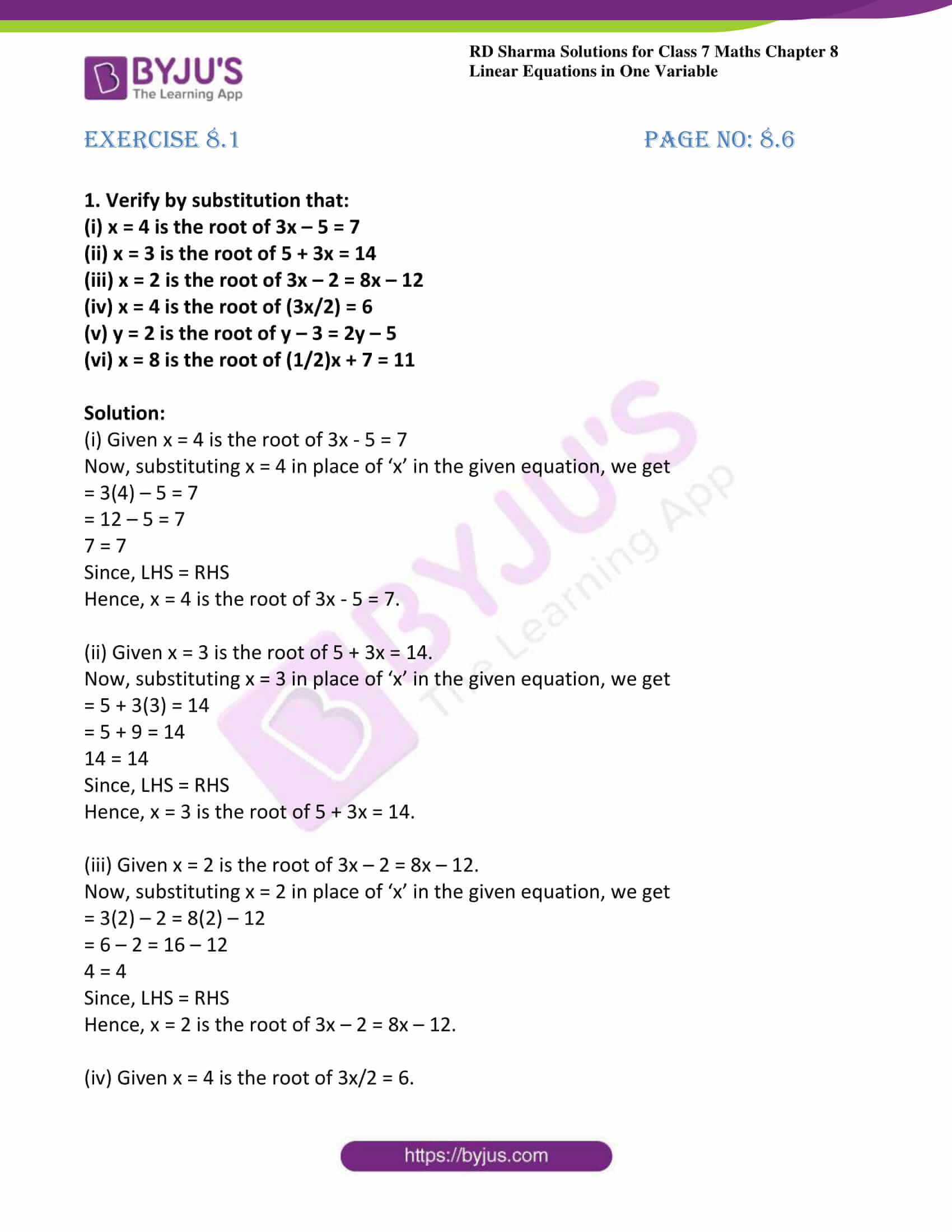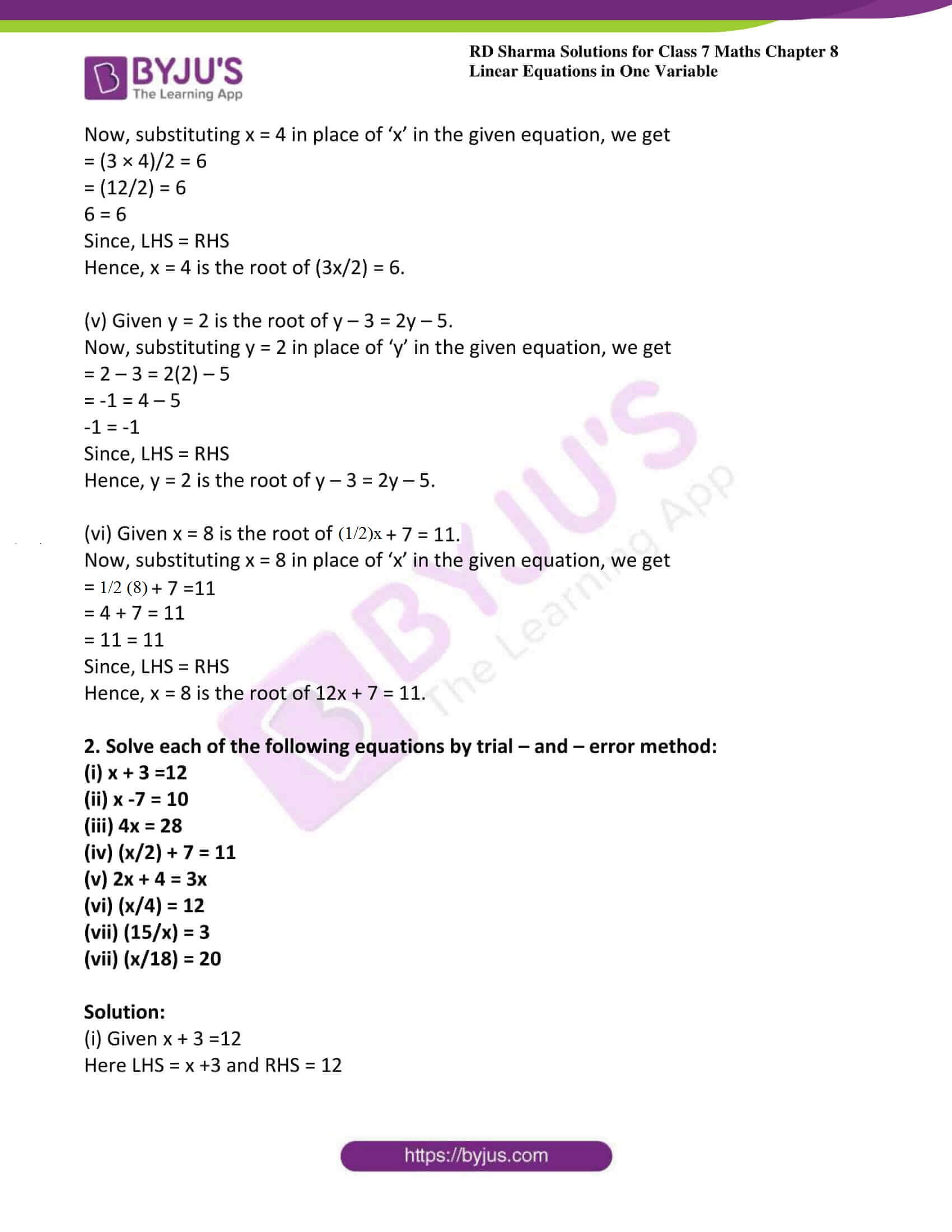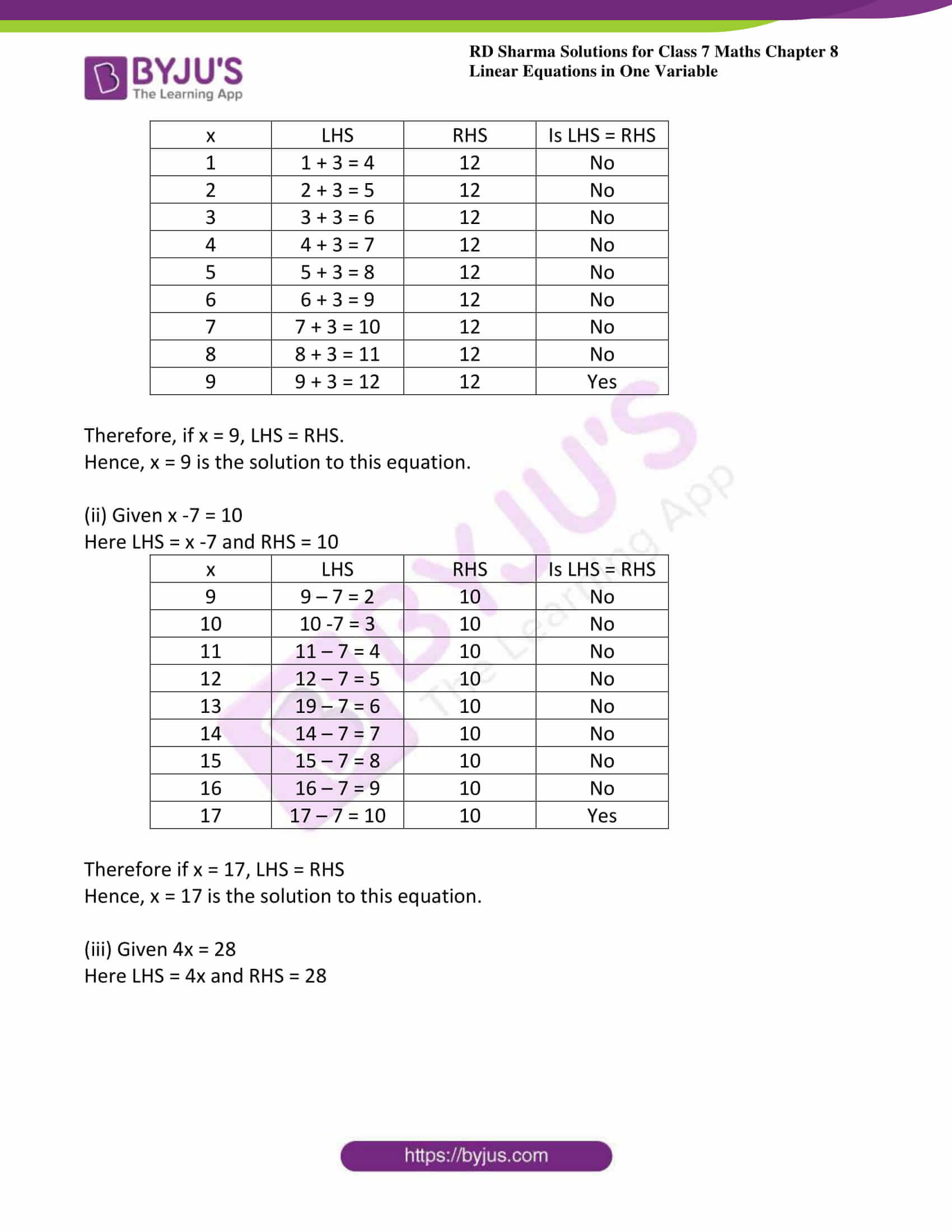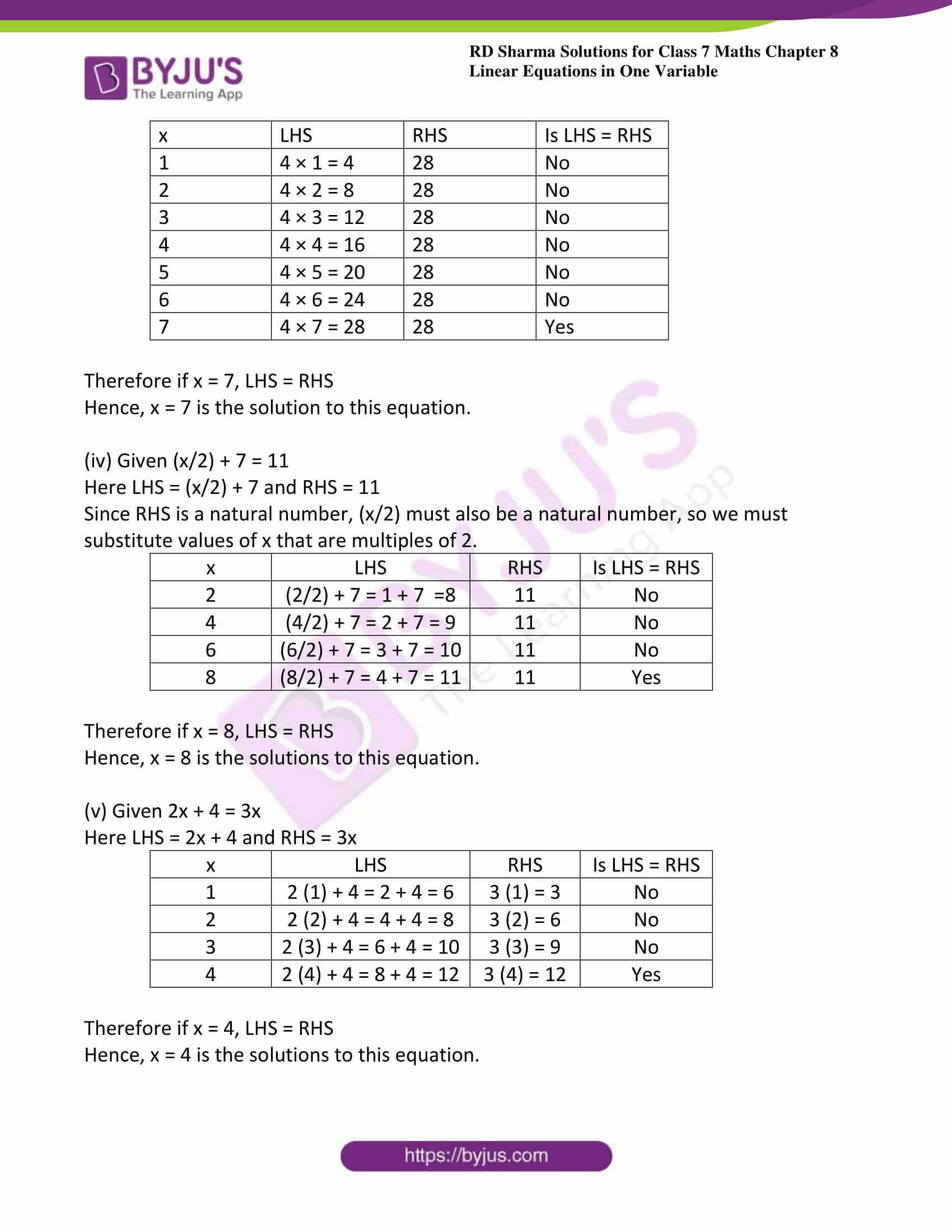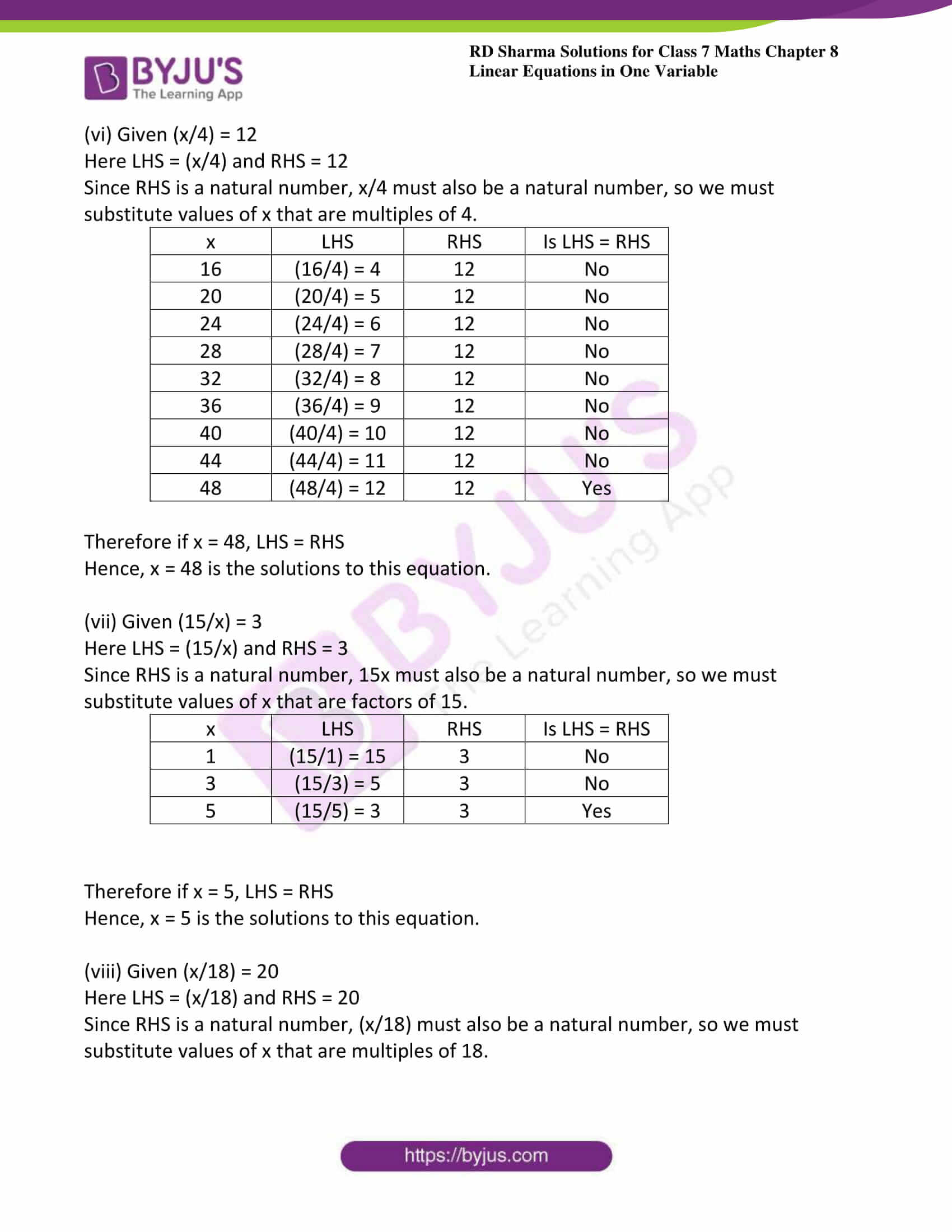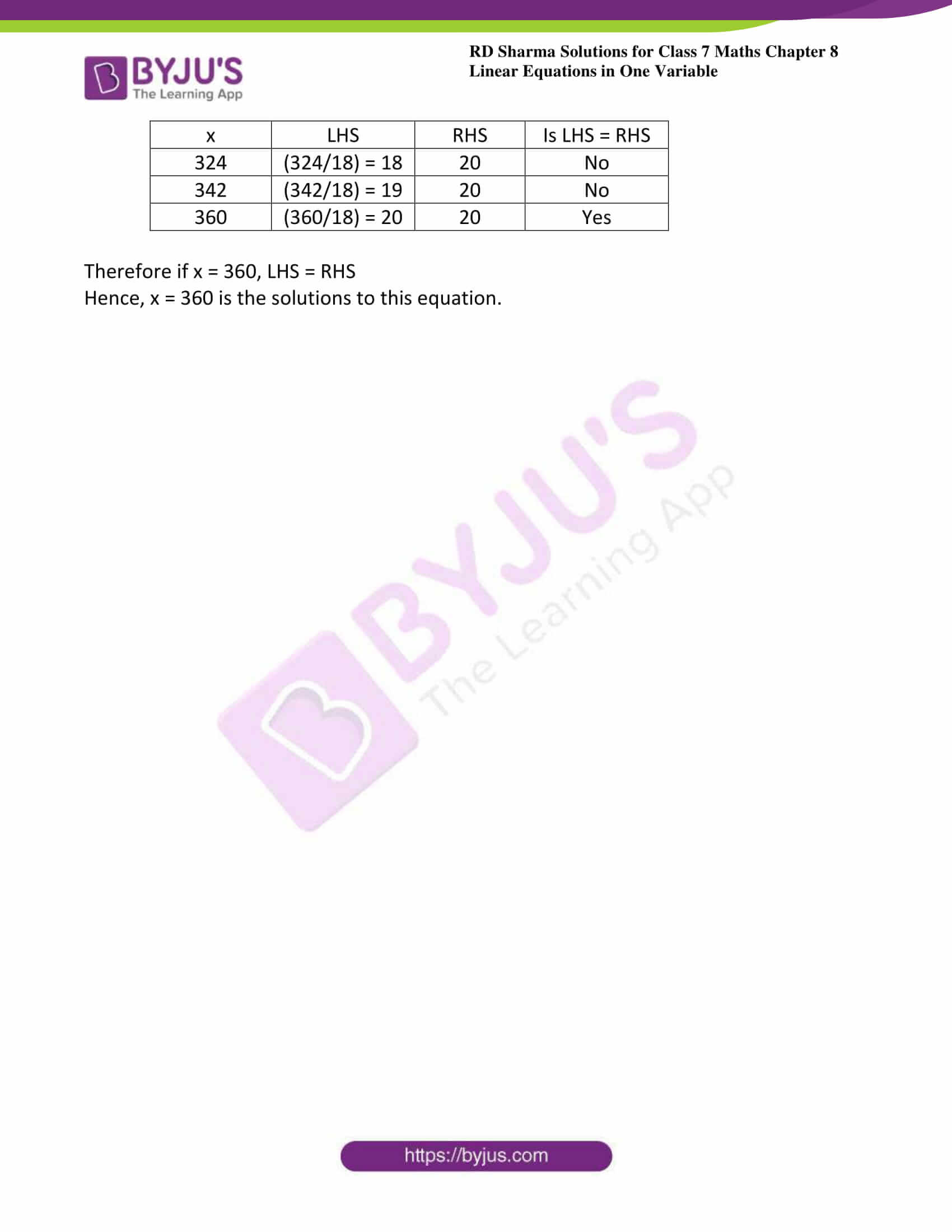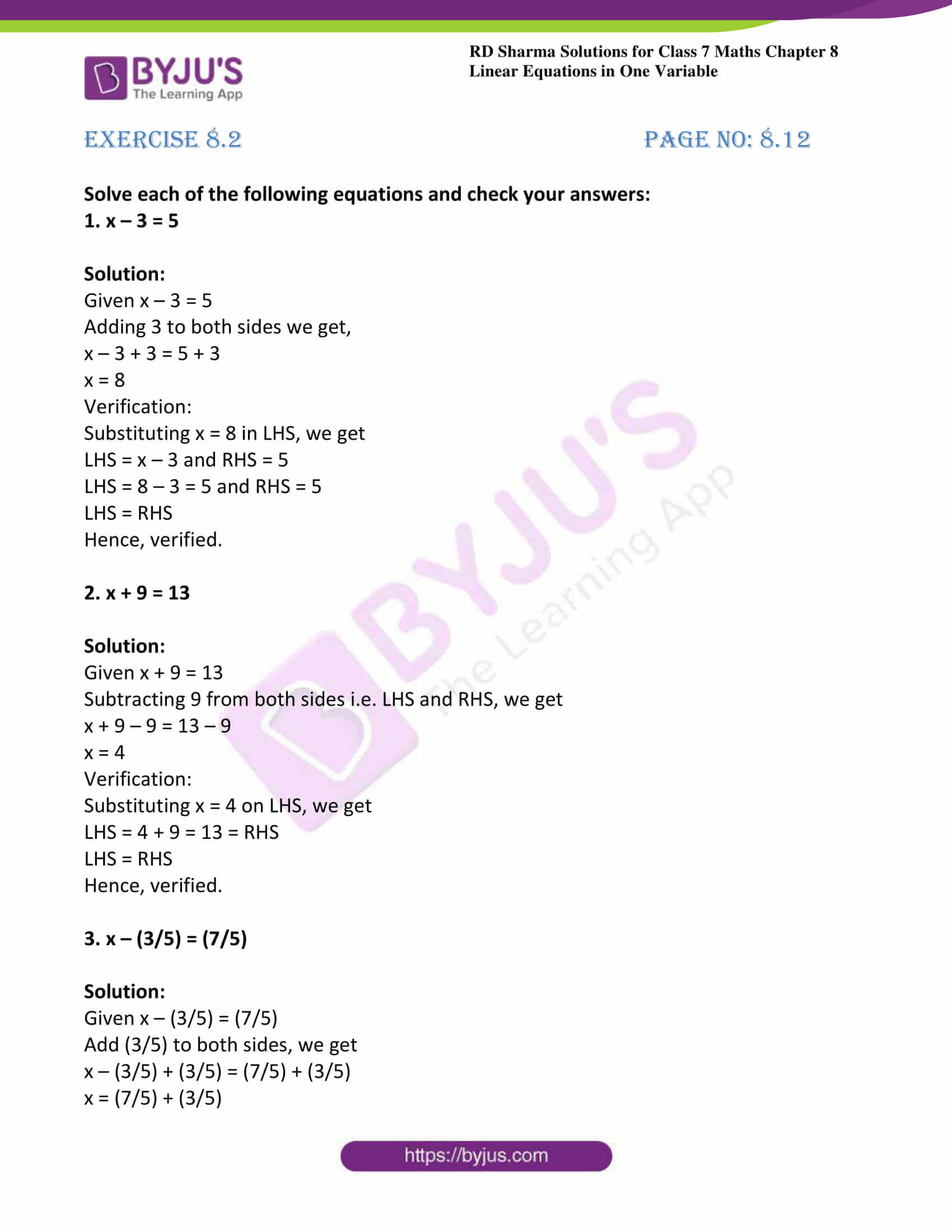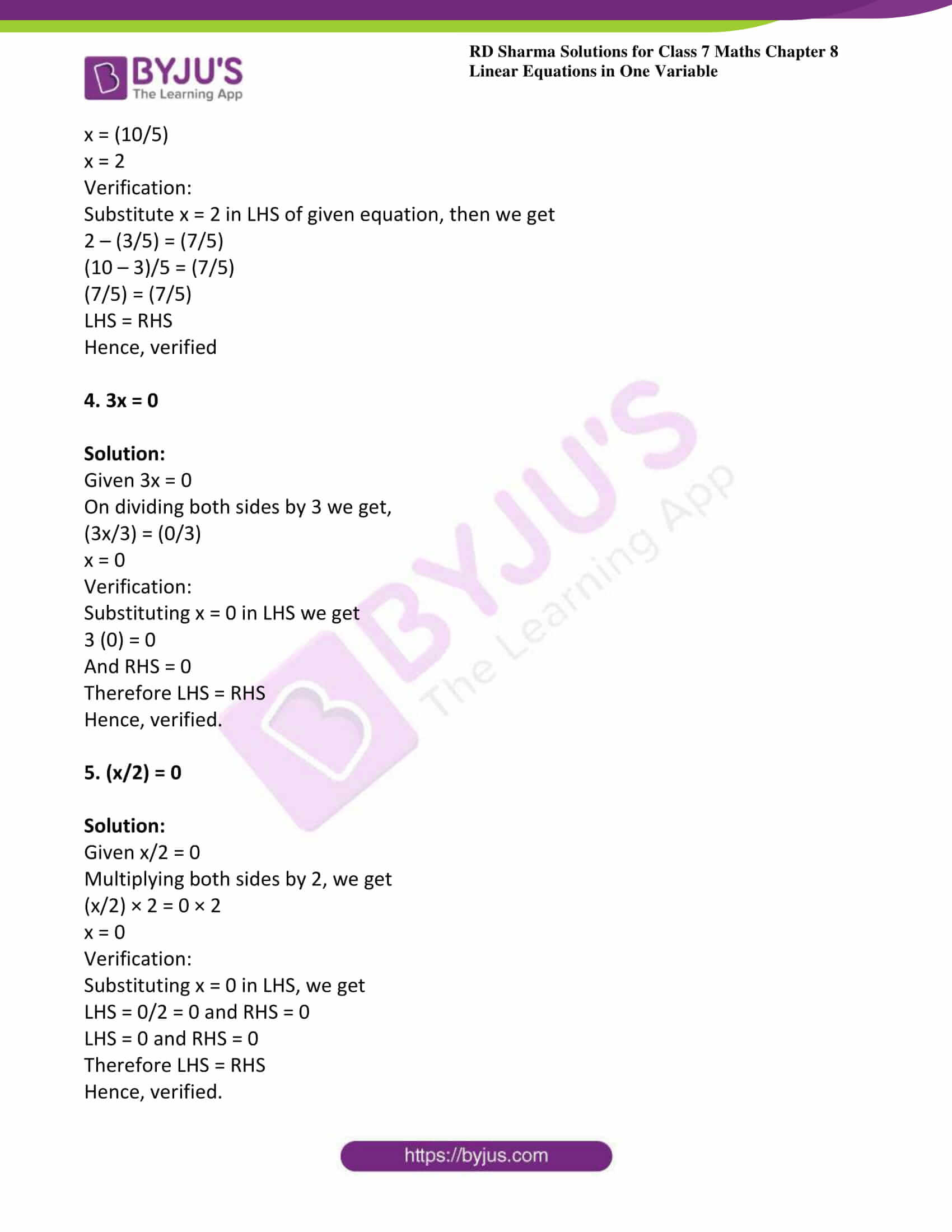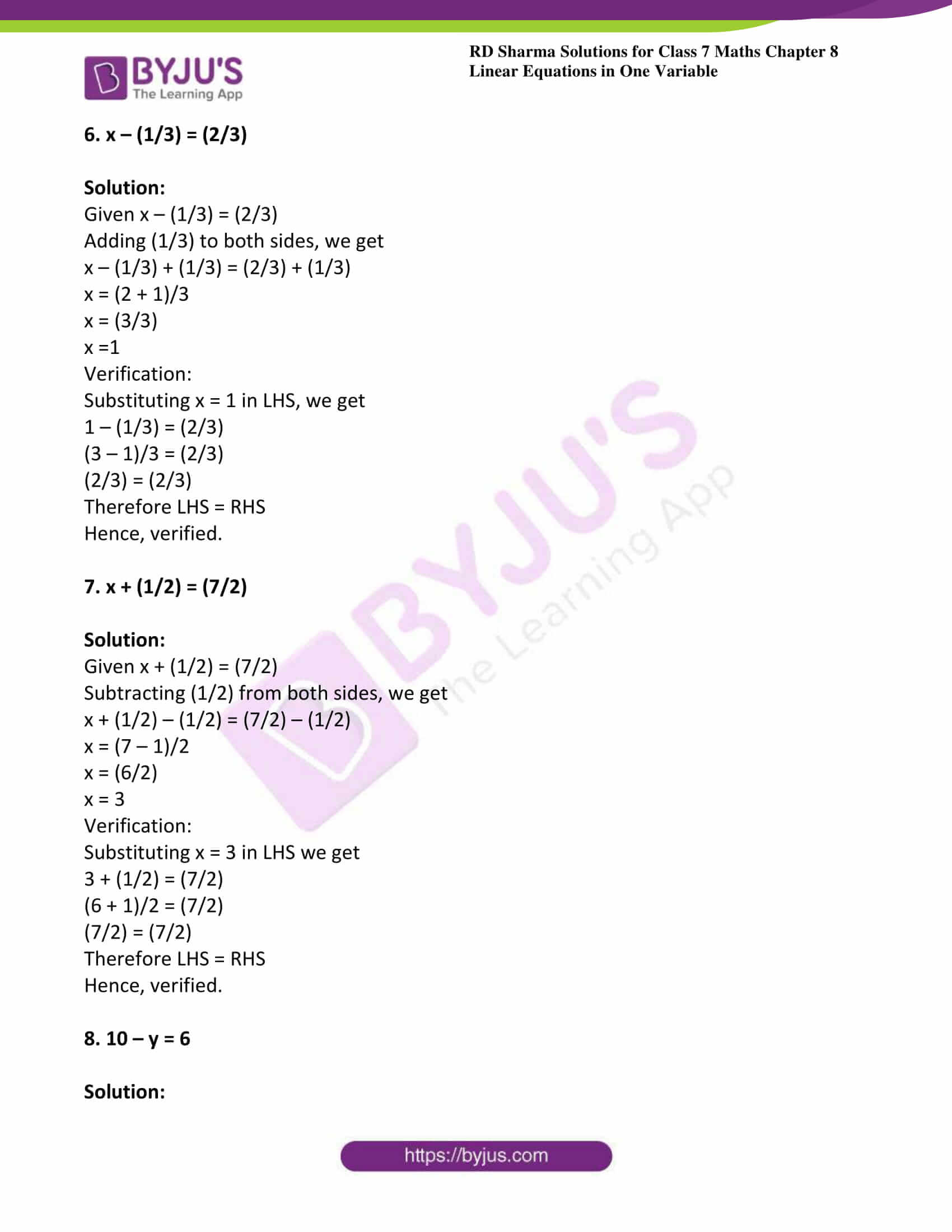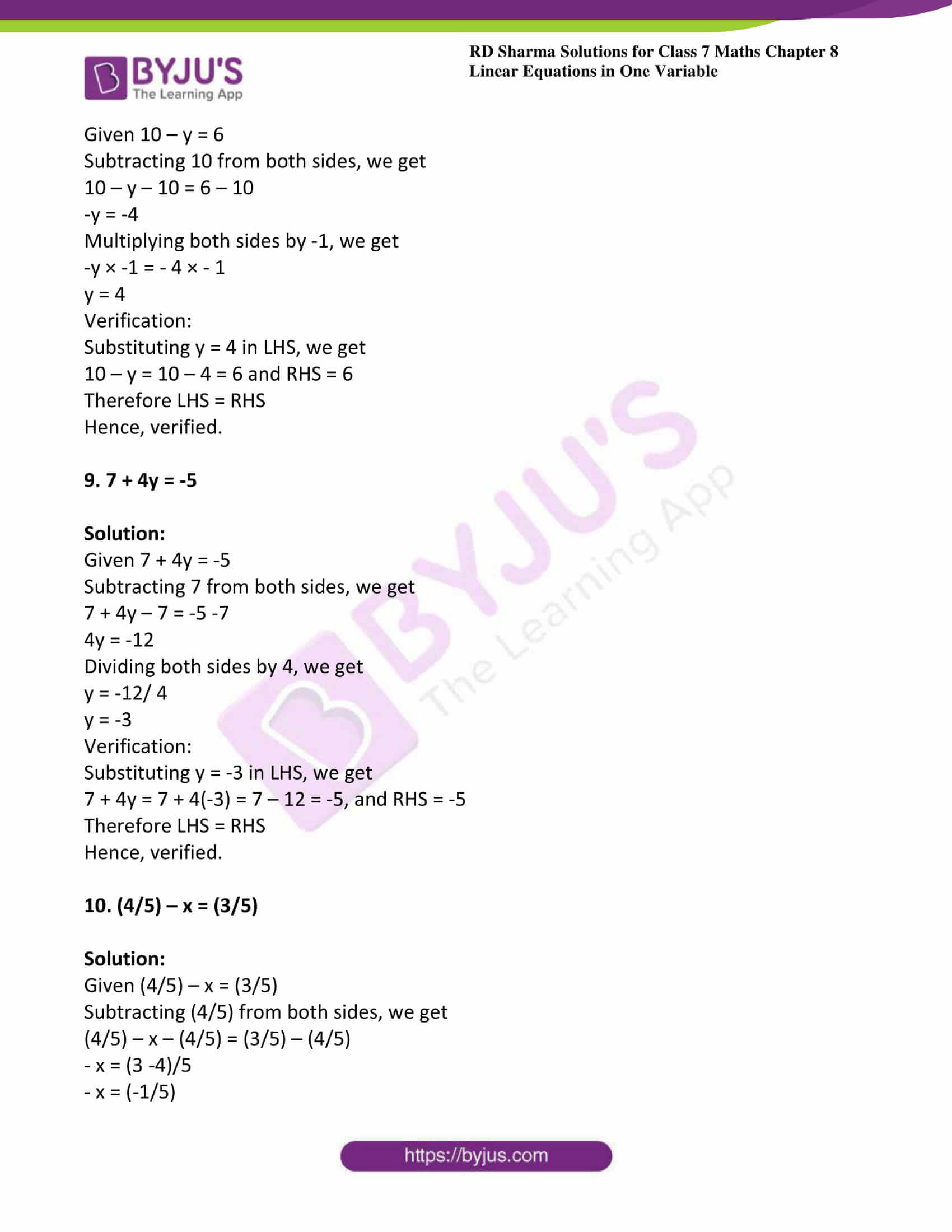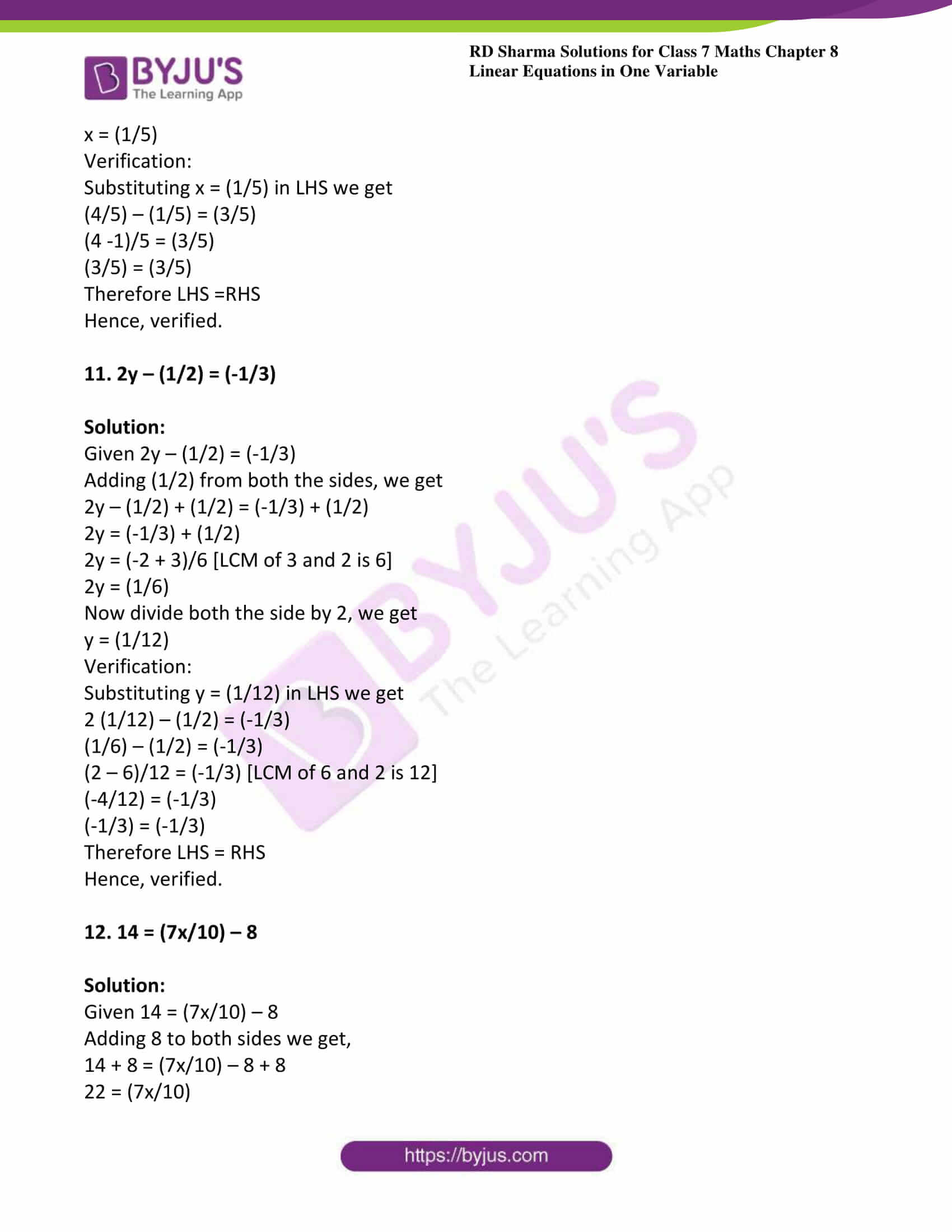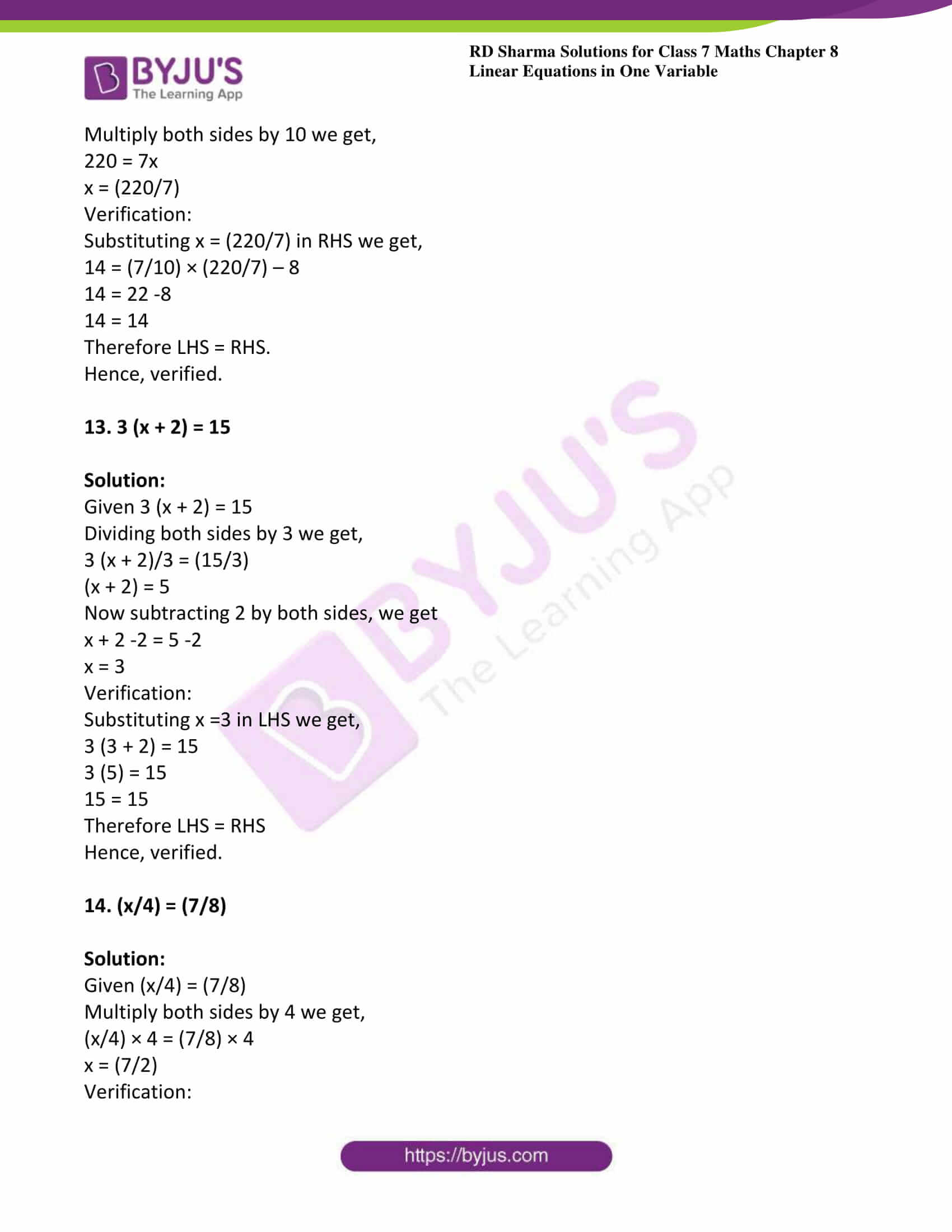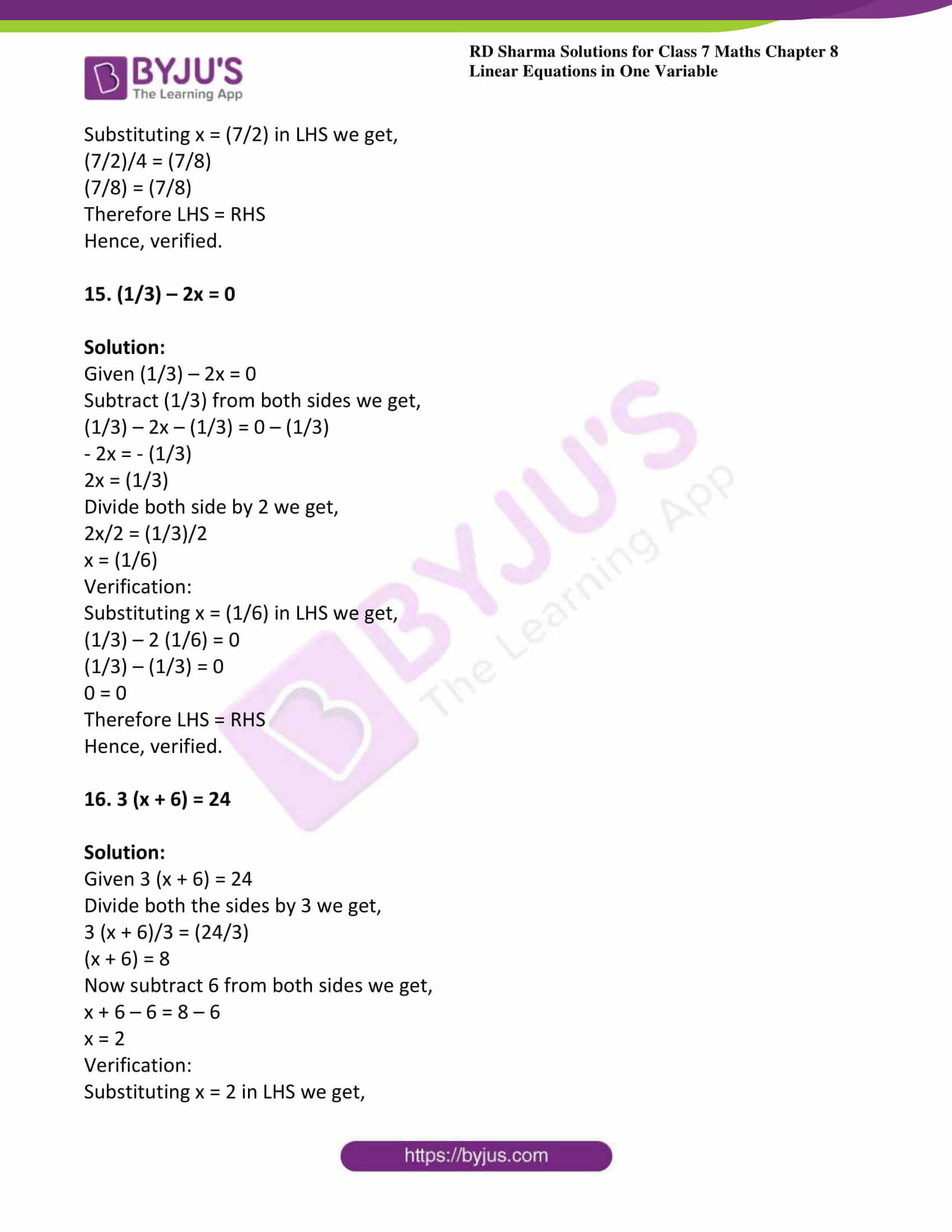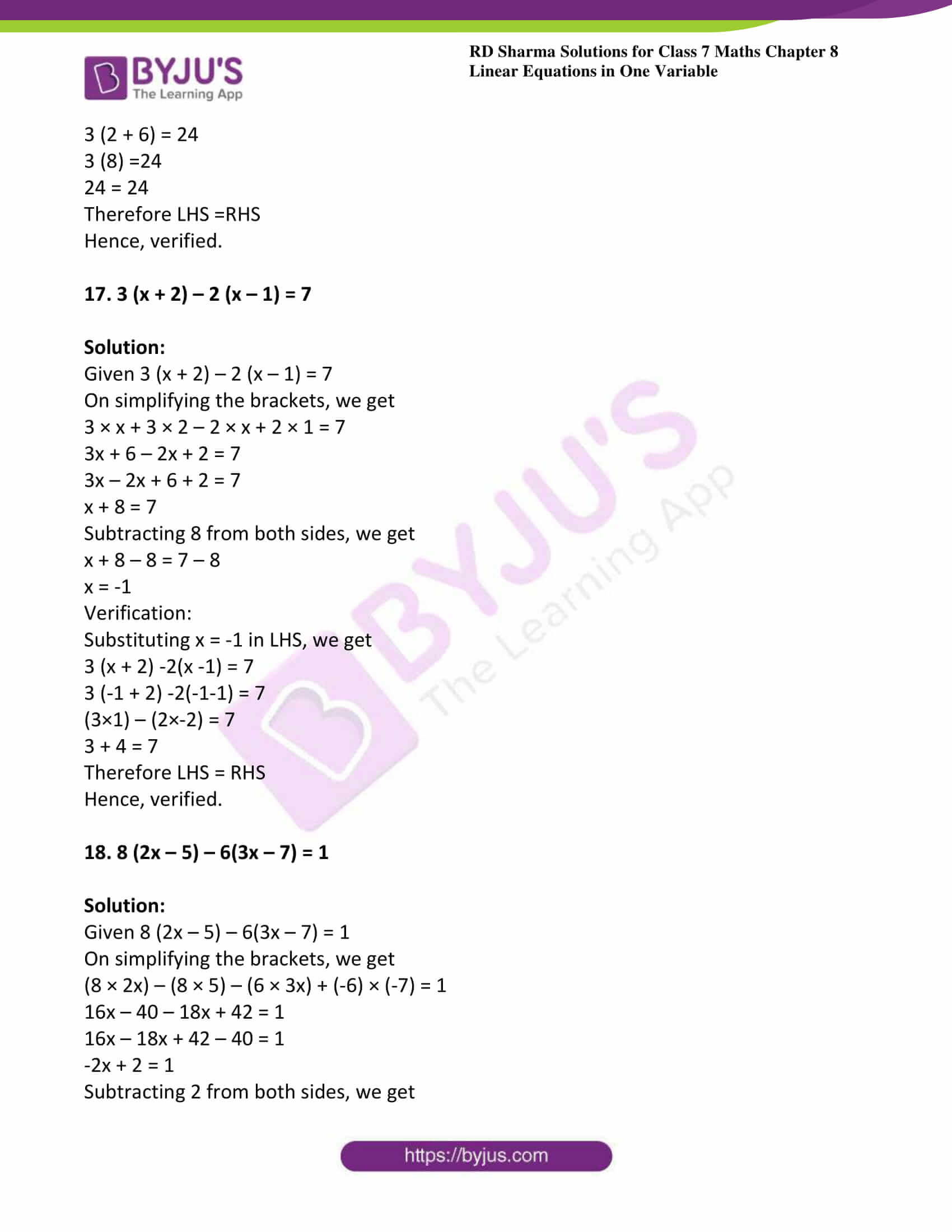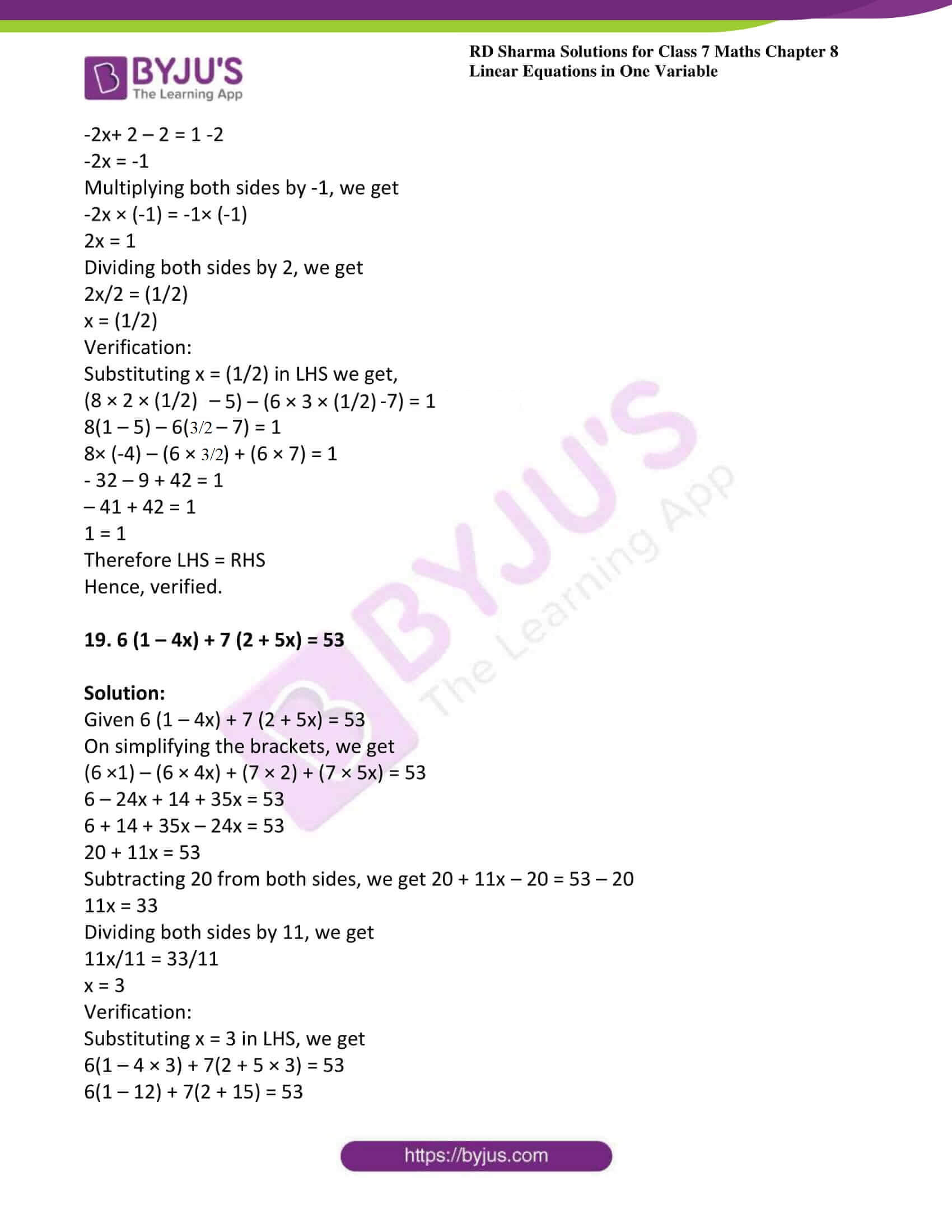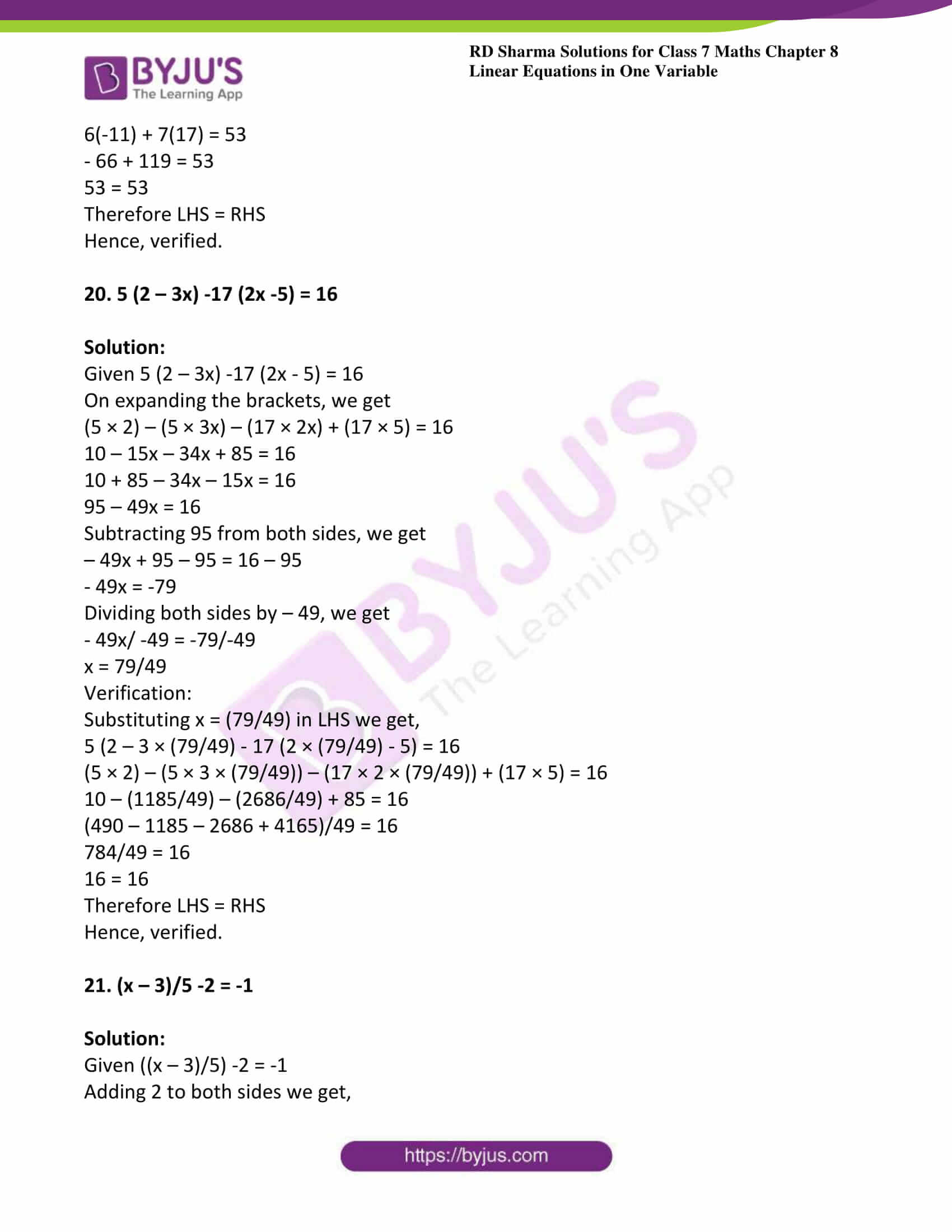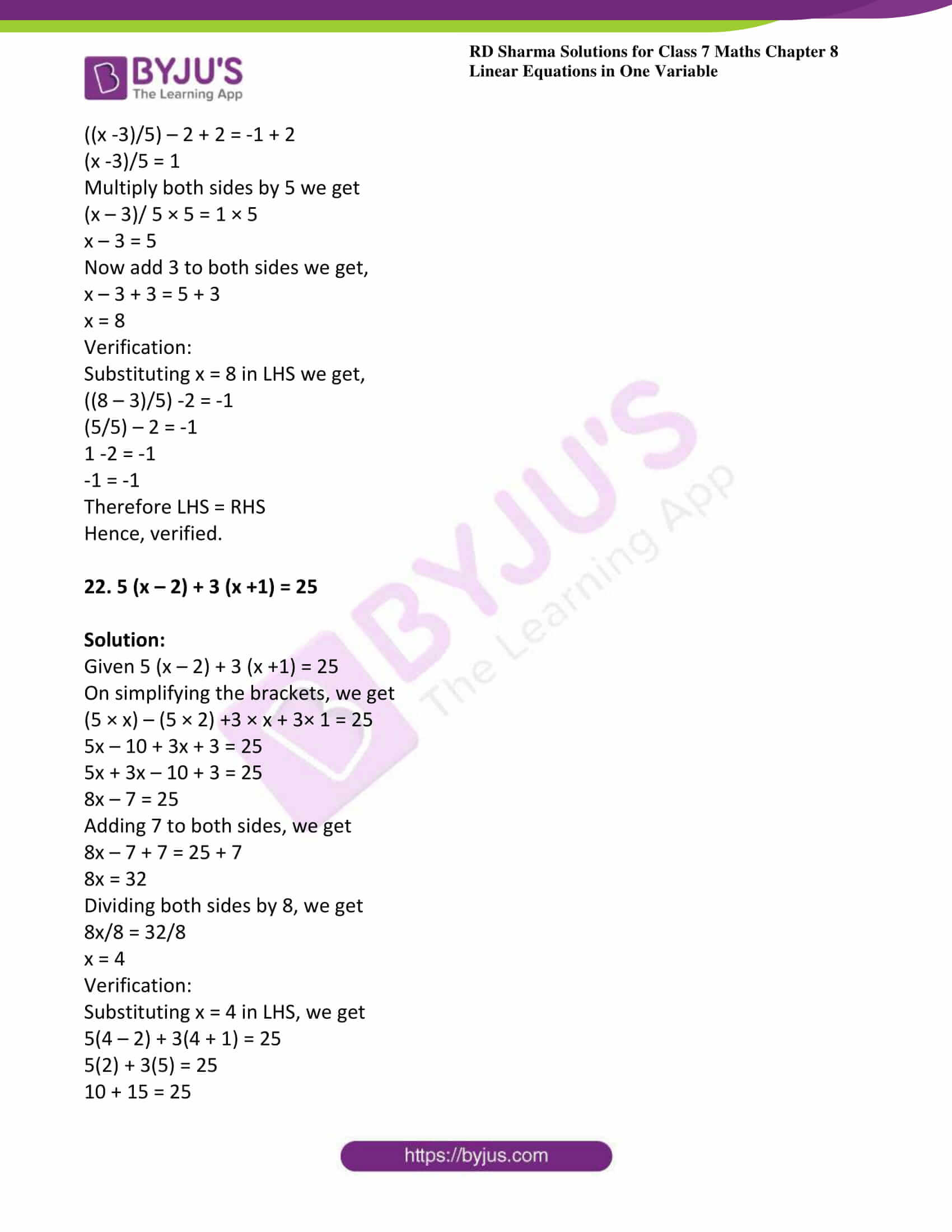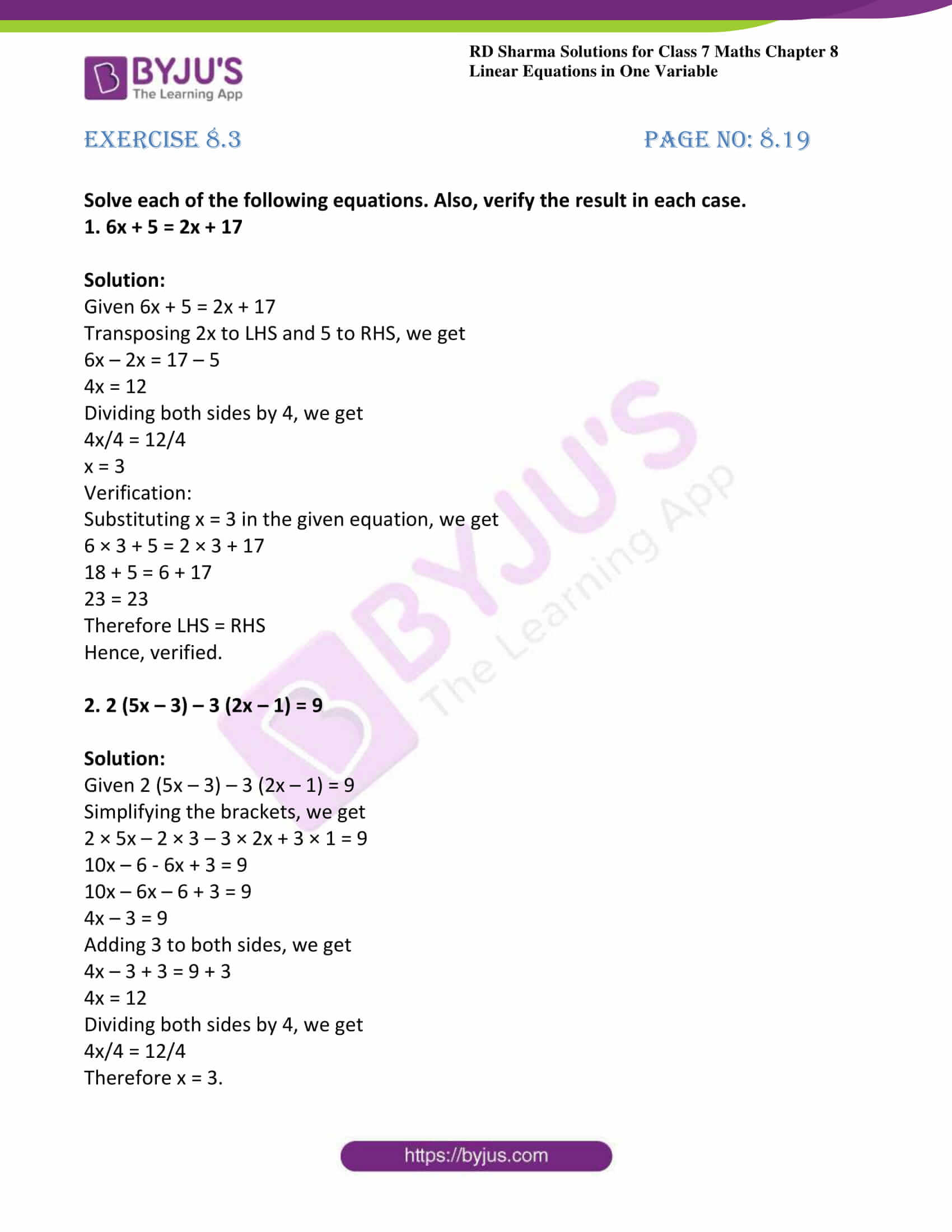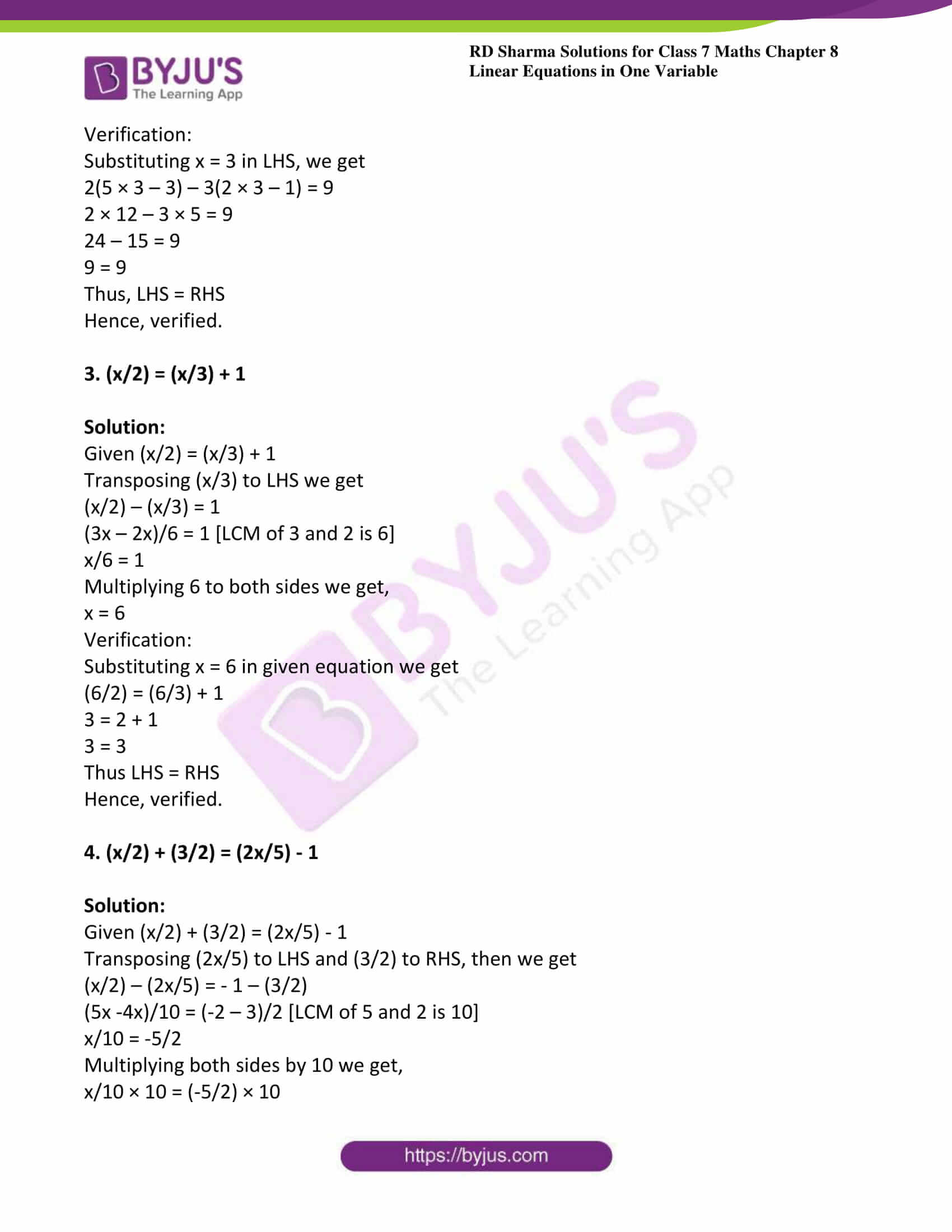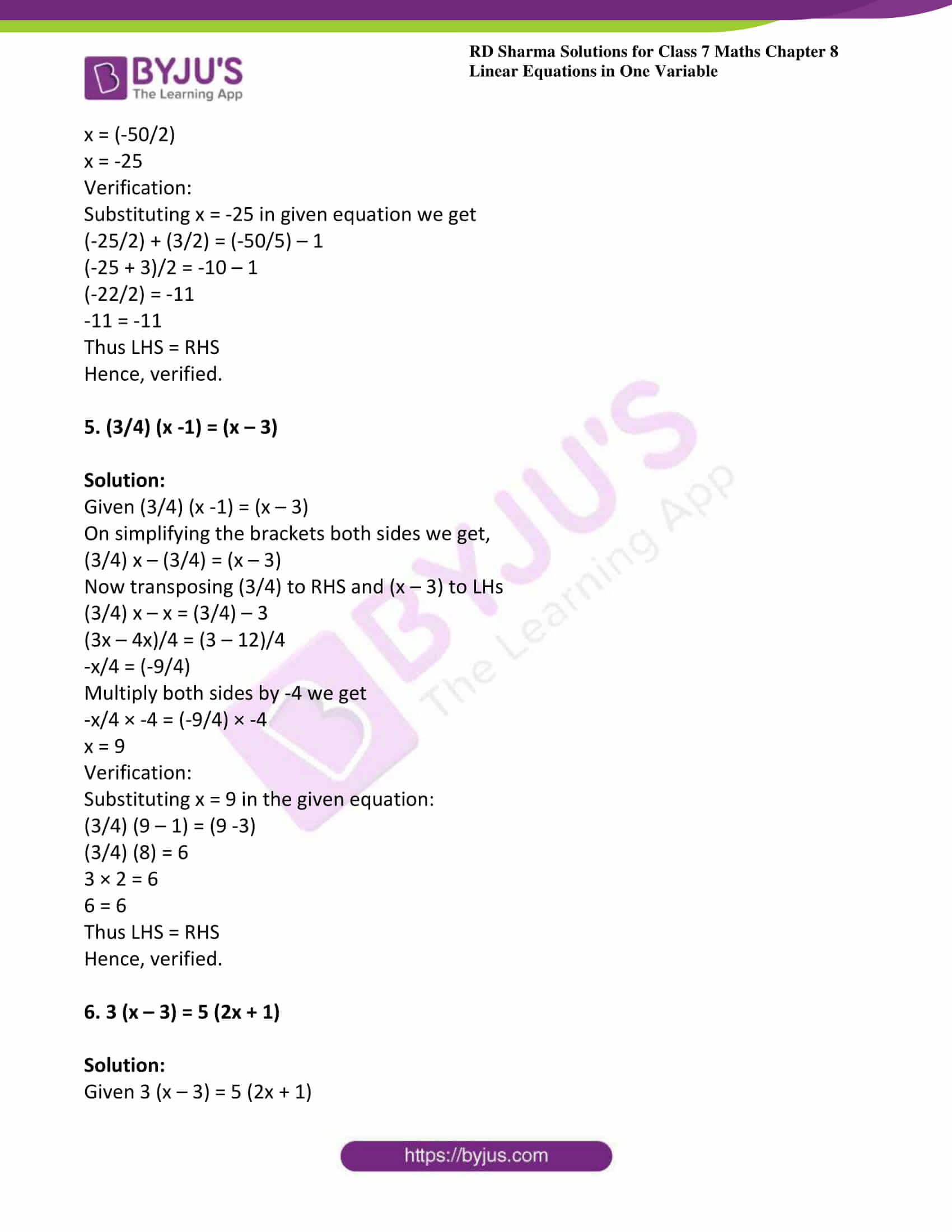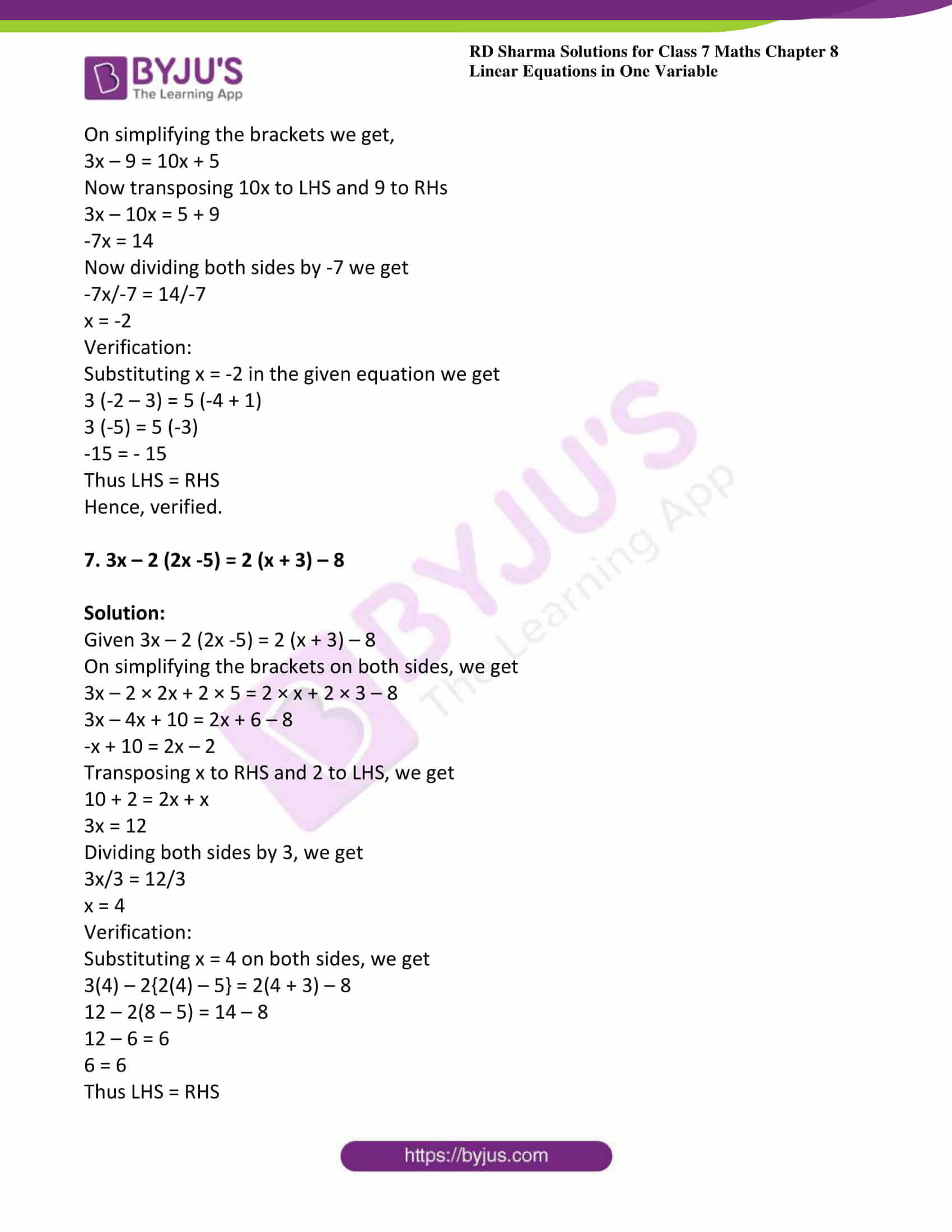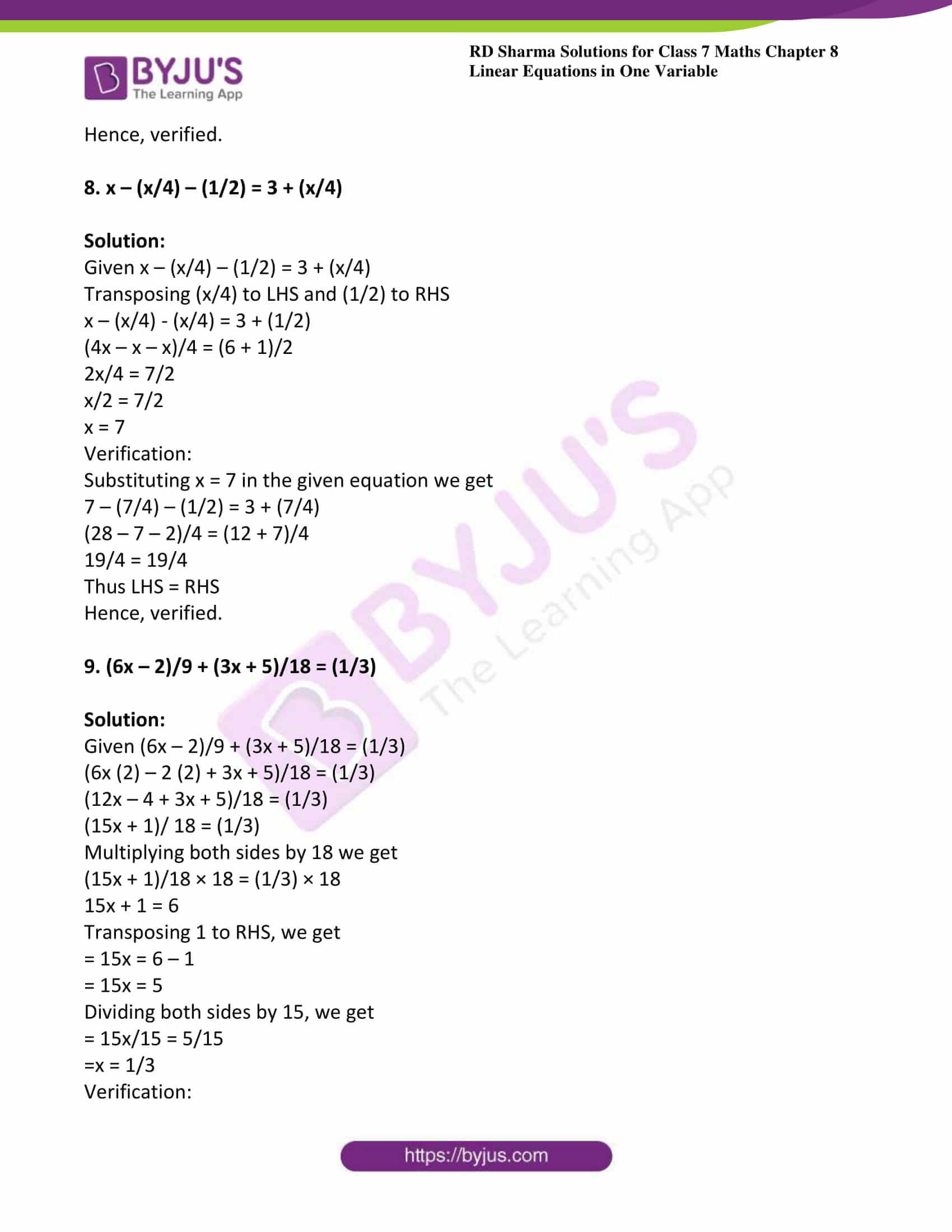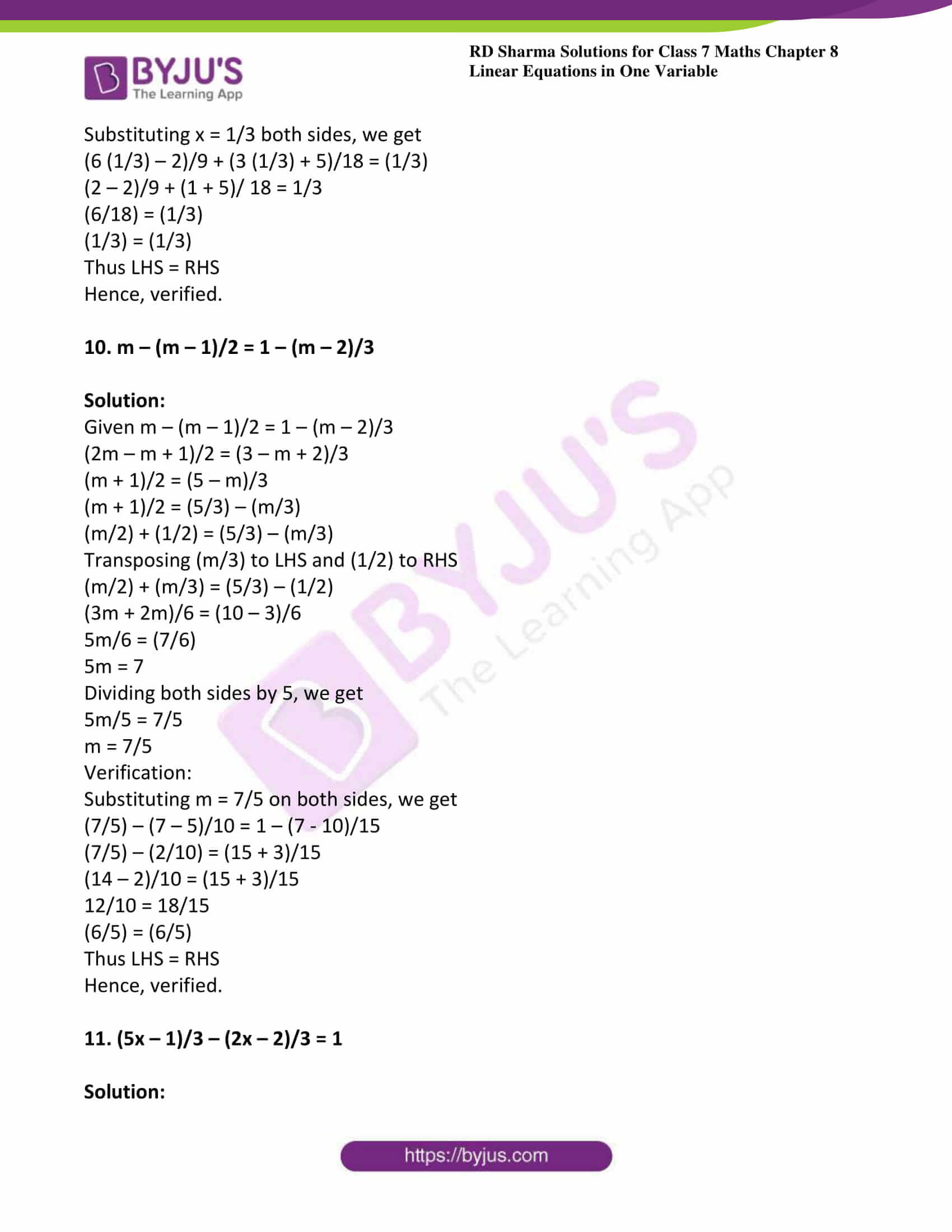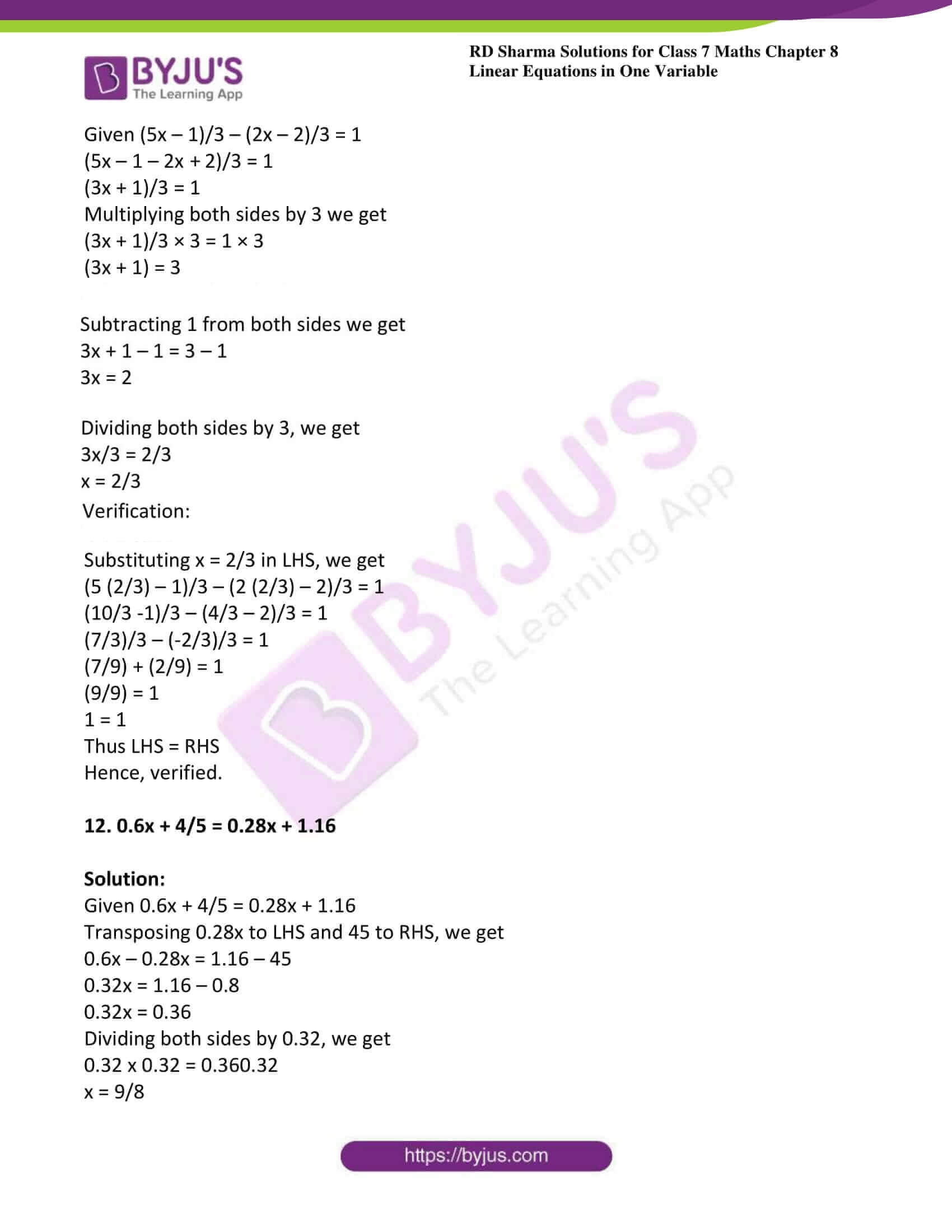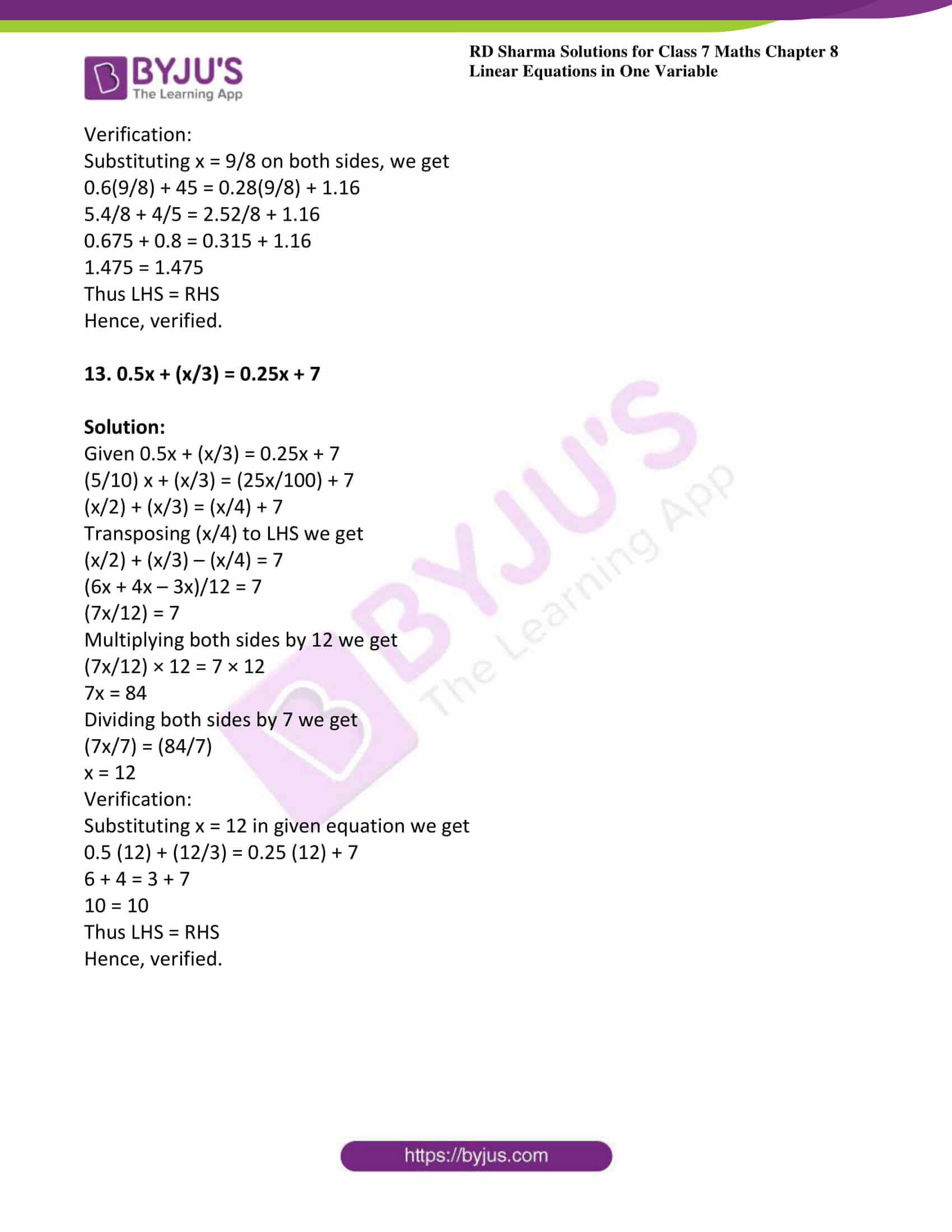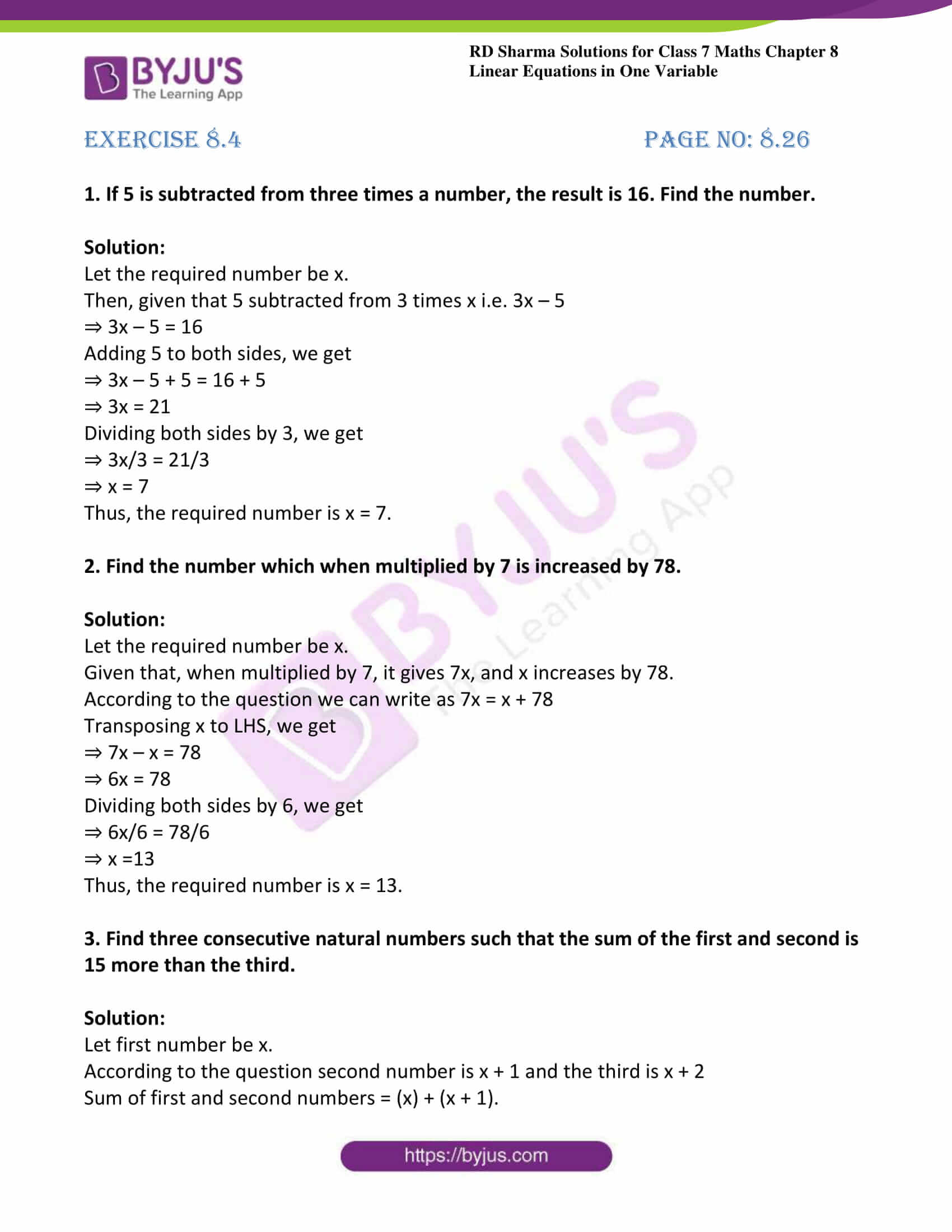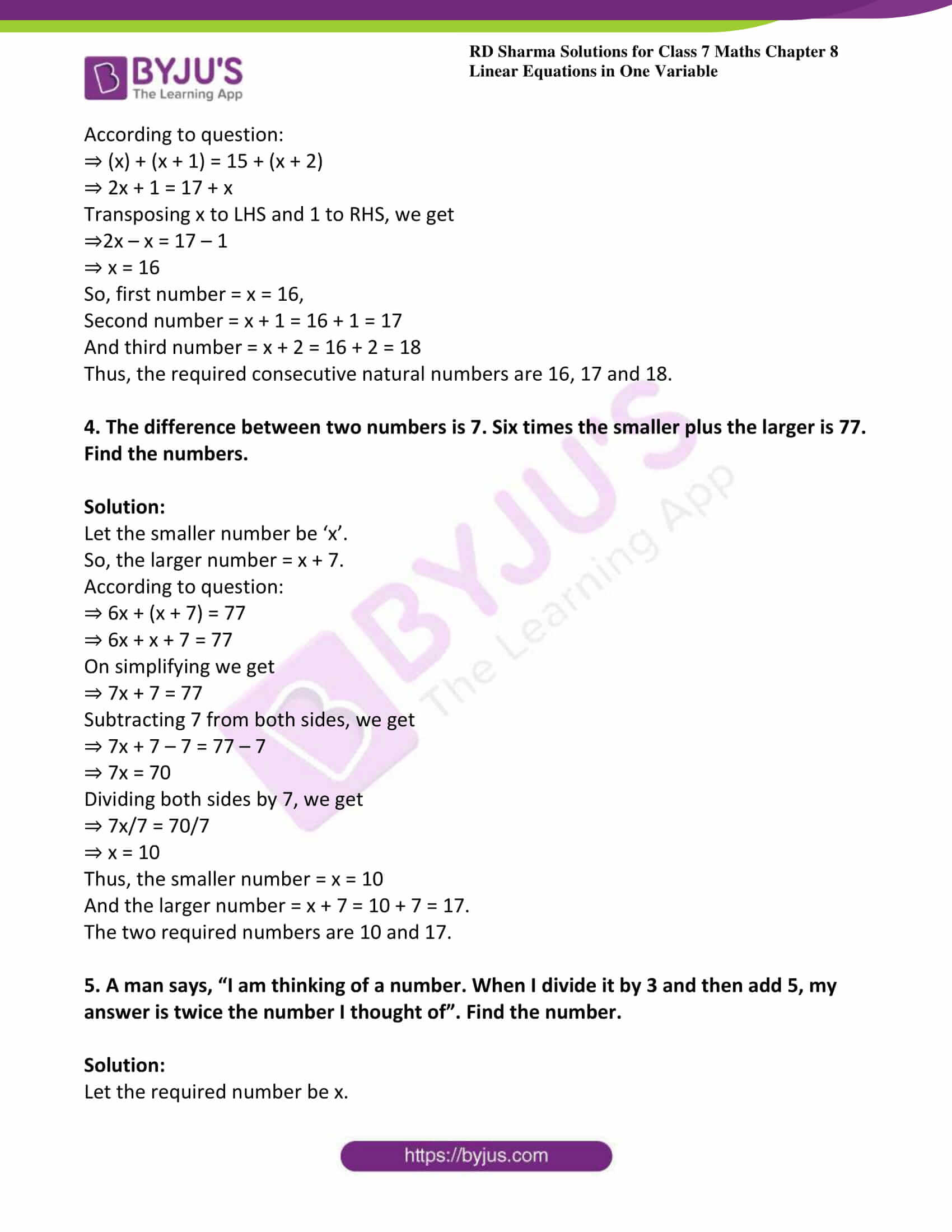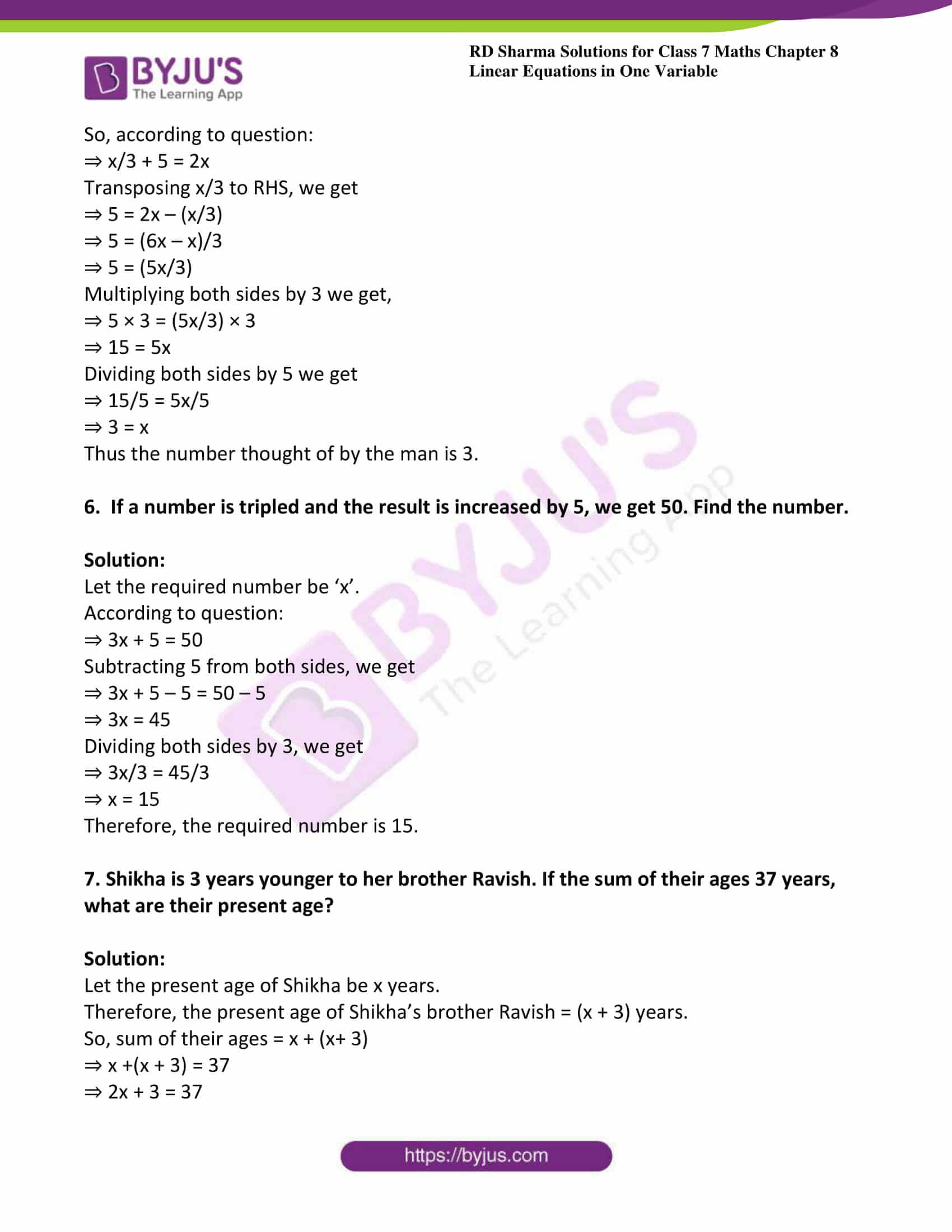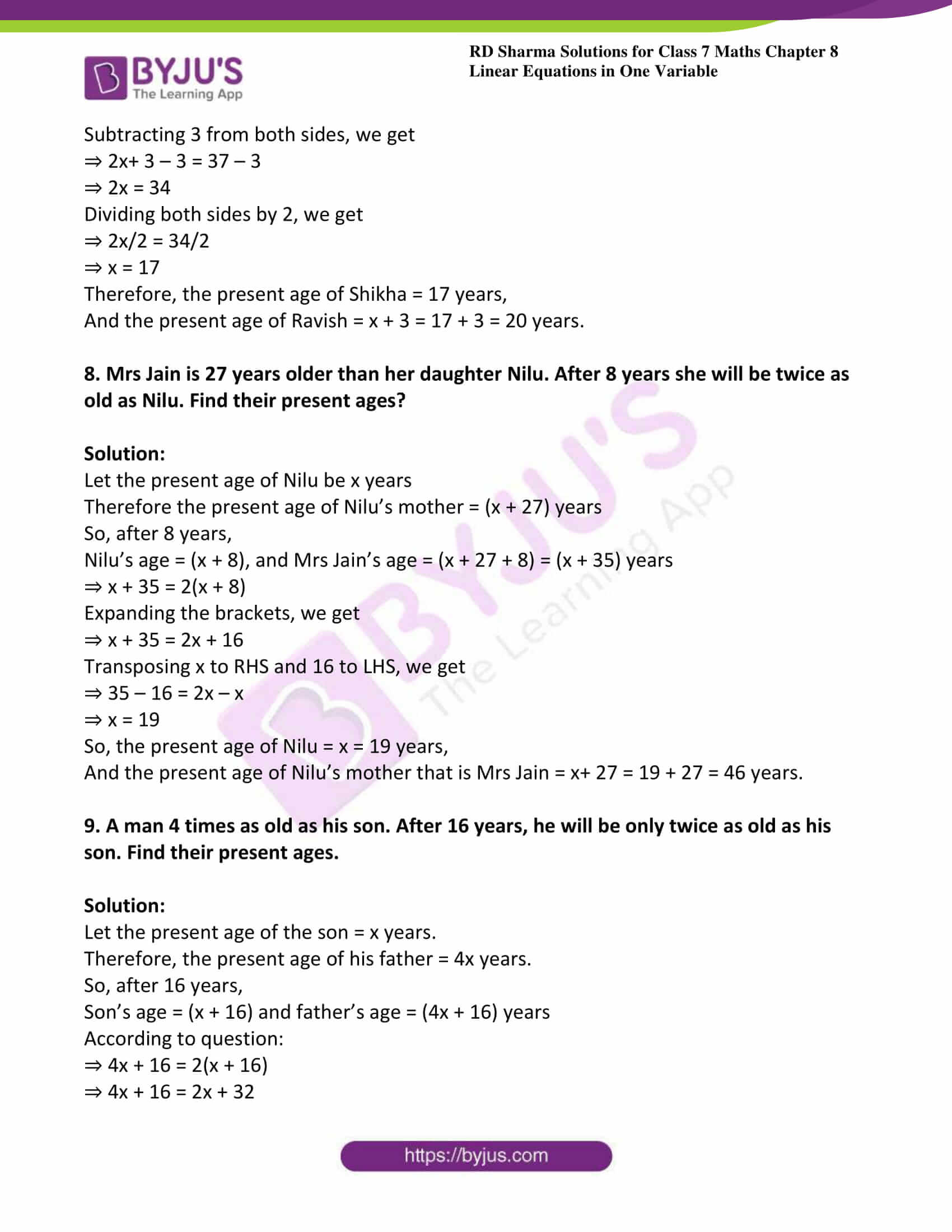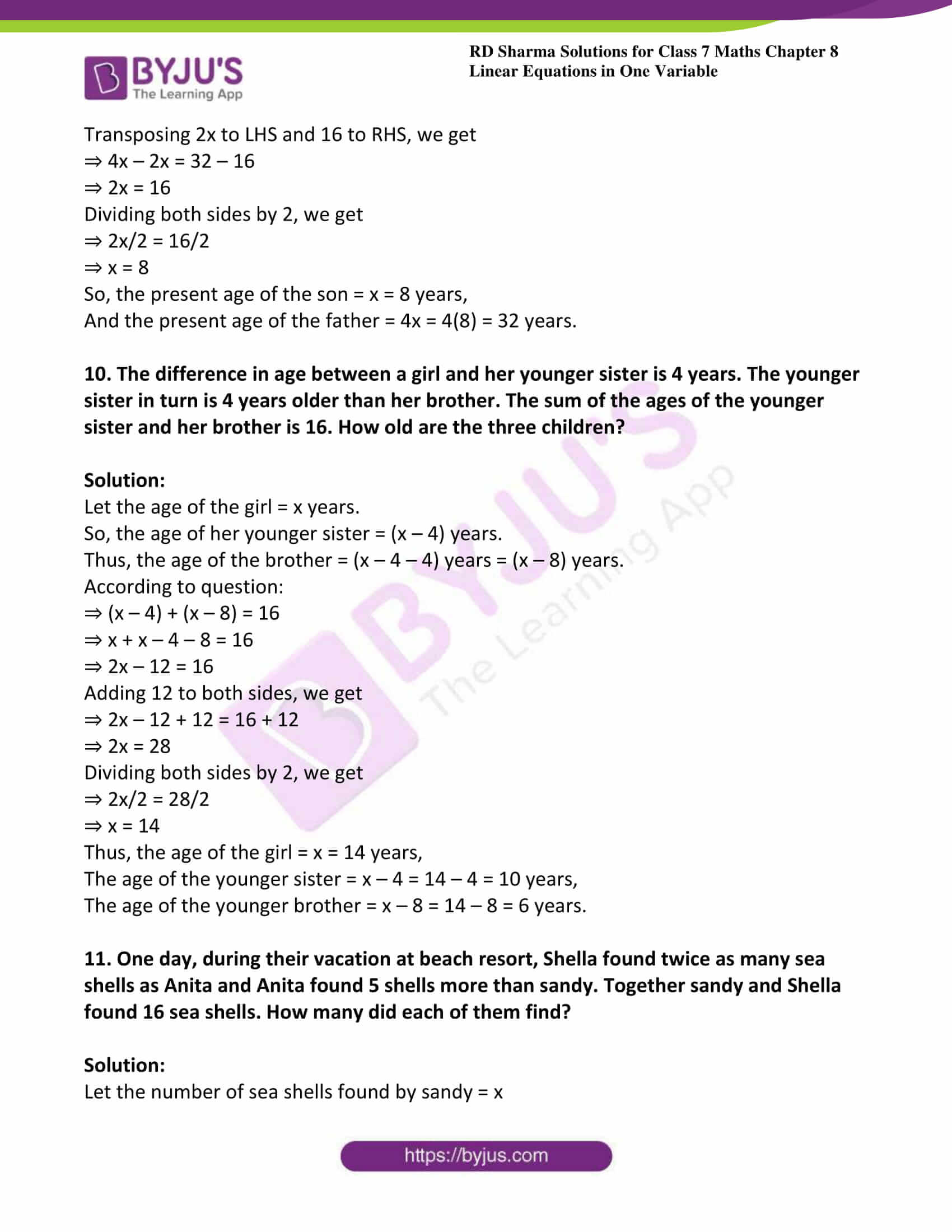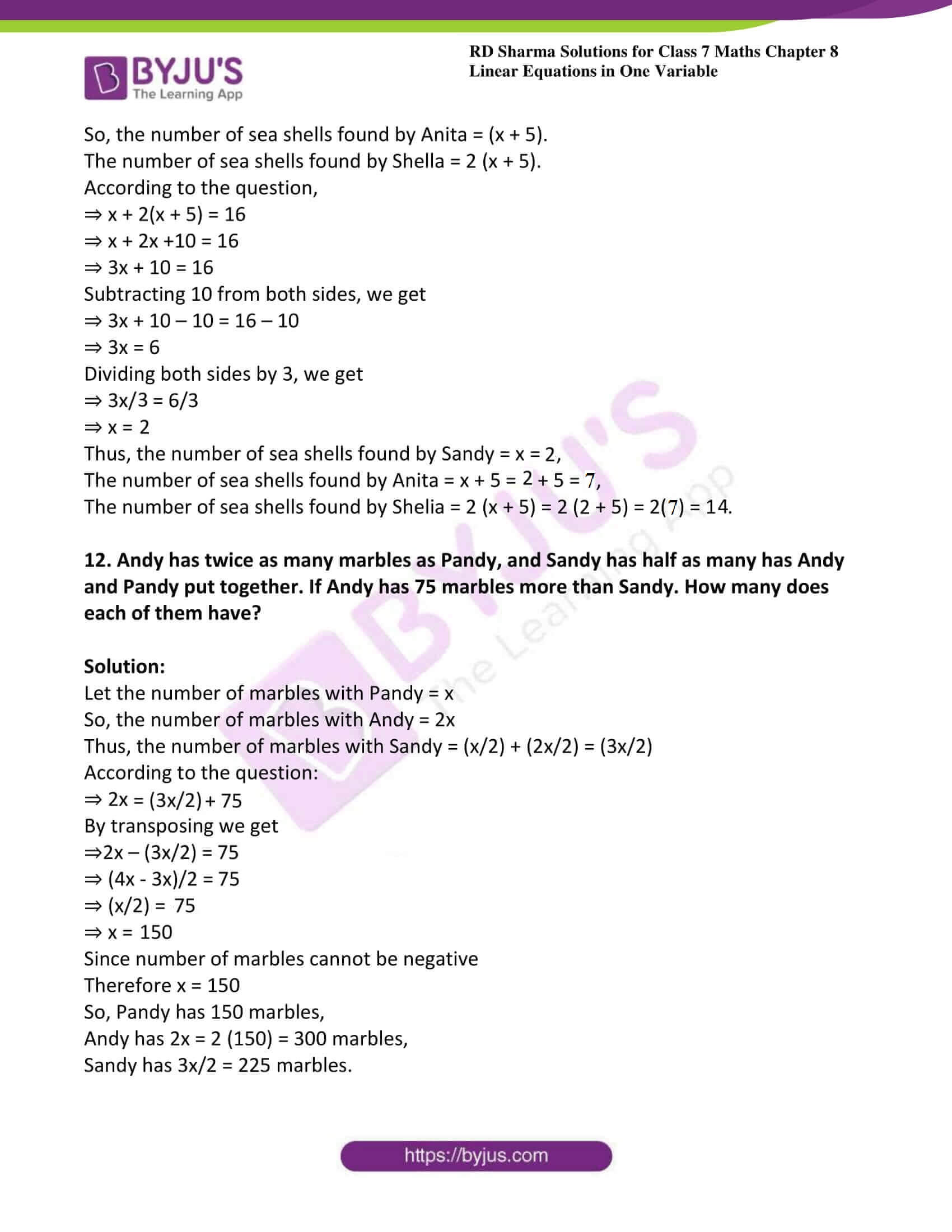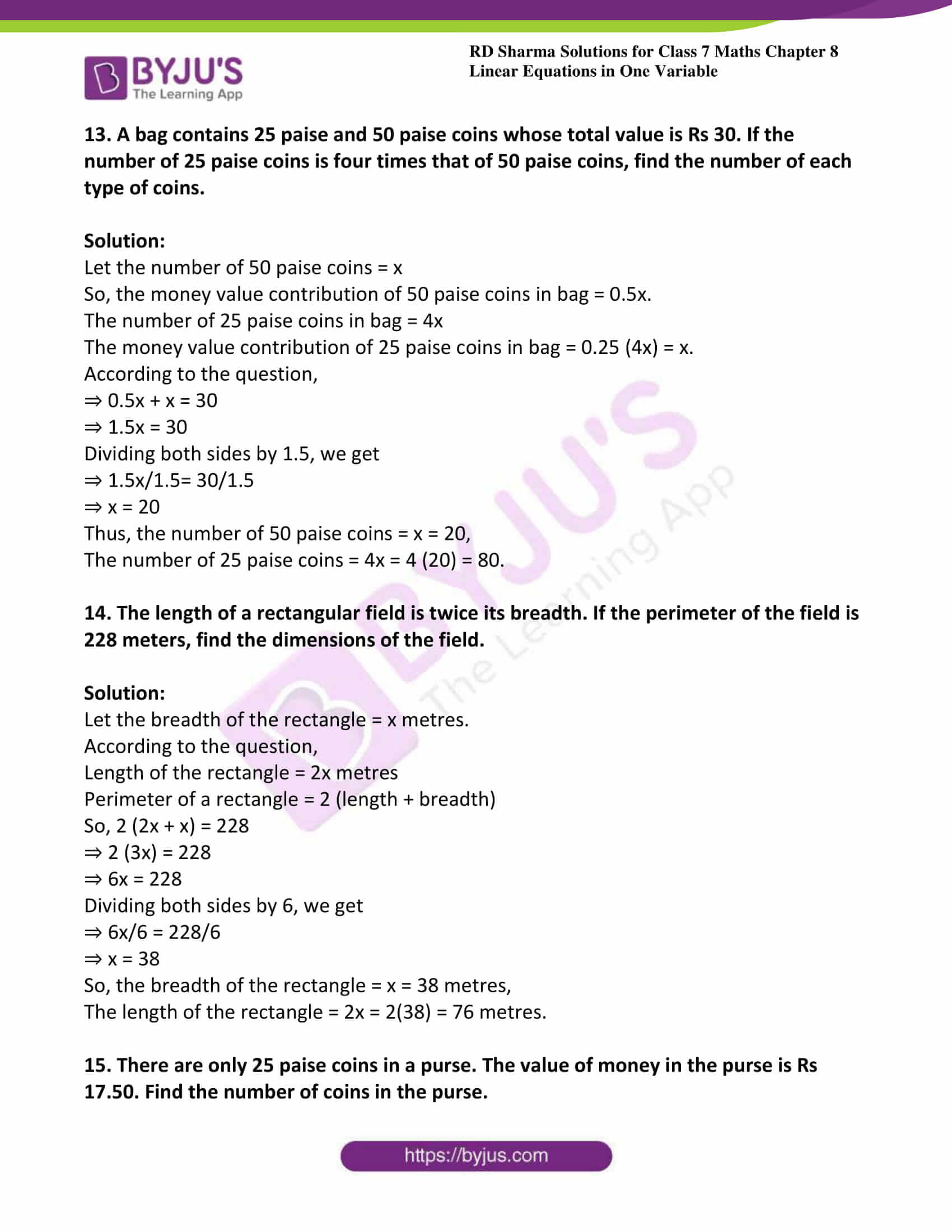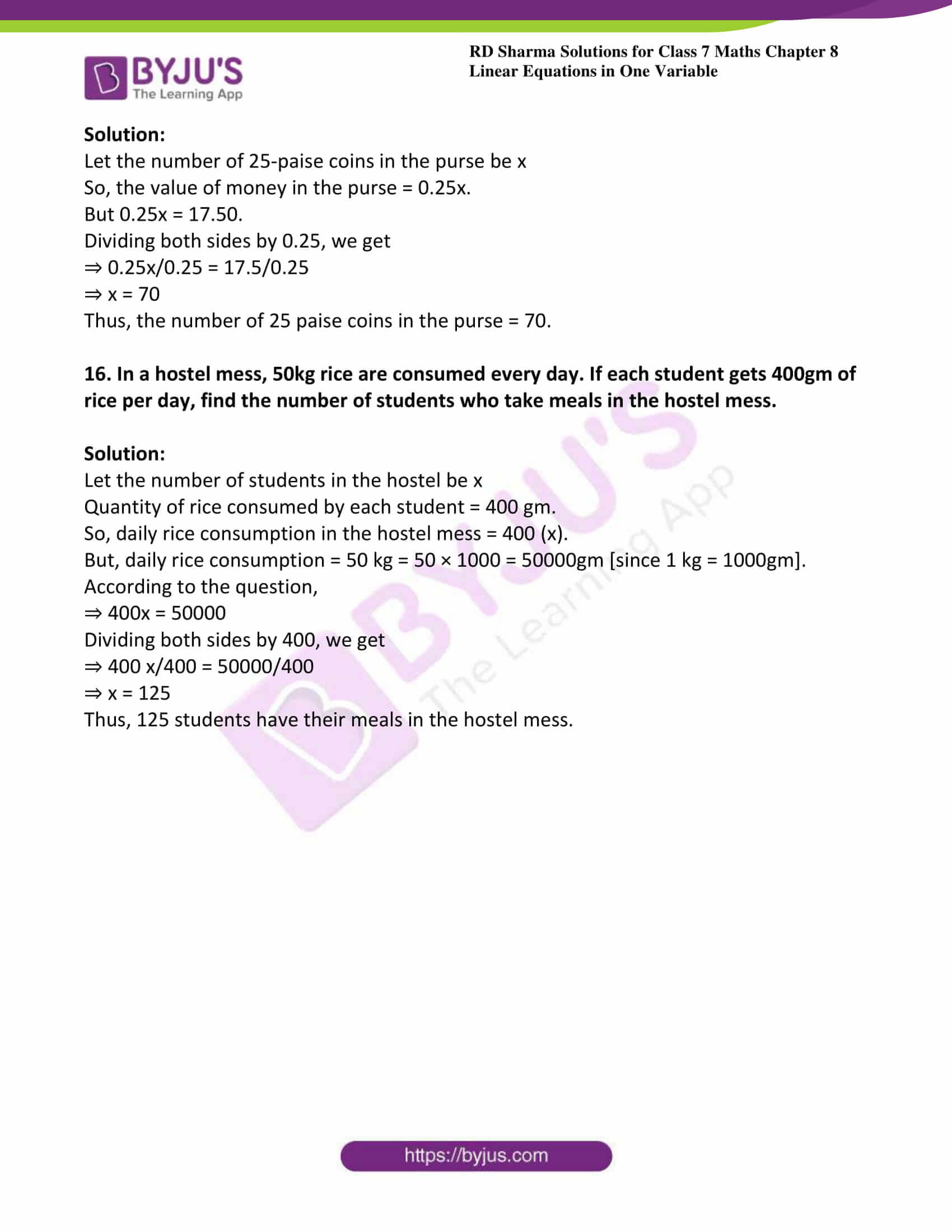### Access answers to Maths RD Sharma Solutions For Class 7 Chapter 8 – Linear Equations in One variable

Exercise 8.1 Page No: 8.6

1. Verify by substitution that:

(i) x = 4 is the root of 3x – 5 = 7

(ii) x = 3 is the root of 5 + 3x = 14

(iii) x = 2 is the root of 3x – 2 = 8x – 12

(iv) x = 4 is the root of (3x/2) = 6

(v) y = 2 is the root of y – 3 = 2y – 5

(vi) x = 8 is the root of (1/2)x + 7 = 11

Solution:

(i) Given x = 4 is the root of 3x – 5 = 7

Now, substituting x = 4 in place of ‘x’ in the given equation, we get

= 3(4) – 5 = 7

= 12 – 5 = 7

7 = 7

Since, LHS = RHS

Hence, x = 4 is the root of 3x – 5 = 7.

(ii) Given x = 3 is the root of 5 + 3x = 14.

Now, substituting x = 3 in place of ‘x’ in the given equation, we get

= 5 + 3(3) = 14

= 5 + 9 = 14

14 = 14

Since, LHS = RHS

Hence, x = 3 is the root of 5 + 3x = 14.

(iii) Given x = 2 is the root of 3x – 2 = 8x – 12.

Now, substituting x = 2 in place of ‘x’ in the given equation, we get

= 3(2) – 2 = 8(2) – 12

= 6 – 2 = 16 – 12

4 = 4

Since, LHS = RHS

Hence, x = 2 is the root of 3x – 2 = 8x – 12.

(iv) Given x = 4 is the root of 3x/2 = 6.

Now, substituting x = 4 in place of ‘x’ in the given equation, we get

= (3 × 4)/2 = 6

= (12/2) = 6

6 = 6

Since, LHS = RHS

Hence, x = 4 is the root of (3x/2) = 6.

(v) Given y = 2 is the root of y – 3 = 2y – 5.

Now, substituting y = 2 in place of ‘y’ in the given equation, we get

= 2 – 3 = 2(2) – 5

= -1 = 4 – 5

-1 = -1

Since, LHS = RHS

Hence, y = 2 is the root of y – 3 = 2y – 5.

(vi) Given x = 8 is the root of (1/2)x + 7 = 11.

Now, substituting x = 8 in place of ‘x’ in the given equation, we get

= (1/2) (8) + 7 =11

= 4 + 7 = 11

= 11 = 11

Since, LHS = RHS

Hence, x = 8 is the root of 12x + 7 = 11.

2. Solve each of the following equations by trial – and – error method:

(i) x + 3 =12

(ii) x -7 = 10

(iii) 4x = 28

(iv) (x/2) + 7 = 11

(v) 2x + 4 = 3x

(vi) (x/4) = 12

(vii) (15/x) = 3

(vii) (x/18) = 20

Solution:

(i) Given x + 3 =12

Here LHS = x +3 and RHS = 12

 x LHS RHS Is LHS = RHS 1 1 + 3 = 4 12 No 2 2 + 3 = 5 12 No 3 3 + 3 = 6 12 No 4 4 + 3 = 7 12 No 5 5 + 3 = 8 12 No 6 6 + 3 = 9 12 No 7 7 + 3 = 10 12 No 8 8 + 3 = 11 12 No 9 9 + 3 = 12 12 Yes

Therefore, if x = 9, LHS = RHS.

Hence, x = 9 is the solution to this equation.

(ii) Given x -7 = 10

Here LHS = x -7 and RHS = 10

 x LHS RHS Is LHS = RHS 9 9 – 7 = 2 10 No 10 10 -7 = 3 10 No 11 11 – 7 = 4 10 No 12 12 – 7 = 5 10 No 13 19 – 7 = 6 10 No 14 14 – 7 = 7 10 No 15 15 – 7 = 8 10 No 16 16 – 7 = 9 10 No 17 17 – 7 = 10 10 Yes

Therefore if x = 17, LHS = RHS

Hence, x = 17 is the solution to this equation.

(iii) Given 4x = 28

Here LHS = 4x and RHS = 28

 x LHS RHS Is LHS = RHS 1 4 × 1 = 4 28 No 2 4 × 2 = 8 28 No 3 4 × 3 = 12 28 No 4 4 × 4 = 16 28 No 5 4 × 5 = 20 28 No 6 4 × 6 = 24 28 No 7 4 × 7 = 28 28 Yes

Therefore if x = 7, LHS = RHS

Hence, x = 7 is the solution to this equation.

(iv) Given (x/2) + 7 = 11

Here LHS = (x/2) + 7 and RHS = 11

Since RHS is a natural number, (x/2) must also be a natural number, so we must substitute values of x that are multiples of 2.

 x LHS RHS Is LHS = RHS 2 (2/2) + 7 = 1 + 7 =8 11 No 4 (4/2) + 7 = 2 + 7 = 9 11 No 6 (6/2) + 7 = 3 + 7 = 10 11 No 8 (8/2) + 7 = 4 + 7 = 11 11 Yes

Therefore if x = 8, LHS = RHS

Hence, x = 8 is the solutions to this equation.

(v) Given 2x + 4 = 3x

Here LHS = 2x + 4 and RHS = 3x

 x LHS RHS Is LHS = RHS 1 2 (1) + 4 = 2 + 4 = 6 3 (1) = 3 No 2 2 (2) + 4 = 4 + 4 = 8 3 (2) = 6 No 3 2 (3) + 4 = 6 + 4 = 10 3 (3) = 9 No 4 2 (4) + 4 = 8 + 4 = 12 3 (4) = 12 Yes

Therefore if x = 4, LHS = RHS

Hence, x = 4 is the solutions to this equation.

(vi) Given (x/4) = 12

Here LHS = (x/4) and RHS = 12

Since RHS is a natural number, x/4 must also be a natural number, so we must substitute values of x that are multiples of 4.

 x LHS RHS Is LHS = RHS 16 (16/4) = 4 12 No 20 (20/4) = 5 12 No 24 (24/4) = 6 12 No 28 (28/4) = 7 12 No 32 (32/4) = 8 12 No 36 (36/4) = 9 12 No 40 (40/4) = 10 12 No 44 (44/4) = 11 12 No 48 (48/4) = 12 12 Yes

Therefore if x = 48, LHS = RHS

Hence, x = 48 is the solutions to this equation.

(vii) Given (15/x) = 3

Here LHS = (15/x) and RHS = 3

Since RHS is a natural number, 15x must also be a natural number, so we must substitute values of x that are factors of 15.

 x LHS RHS Is LHS = RHS 1 (15/1) = 15 3 No 3 (15/3) = 5 3 No 5 (15/5) = 3 3 Yes

Therefore if x = 5, LHS = RHS

Hence, x = 5 is the solutions to this equation.

(viii) Given (x/18) = 20

Here LHS = (x/18) and RHS = 20

Since RHS is a natural number, (x/18) must also be a natural number, so we must substitute values of x that are multiples of 18.

 x LHS RHS Is LHS = RHS 324 (324/18) = 18 20 No 342 (342/18) = 19 20 No 360 (360/18) = 20 20 Yes

Therefore if x = 360, LHS = RHS

Hence, x = 360 is the solutions to this equation.

Exercise 8.2 Page No: 8.12

1. x – 3 = 5

Solution:

Given x – 3 = 5

Adding 3 to both sides we get,

x – 3 + 3 = 5 + 3

x = 8

Verification:

Substituting x = 8 in LHS, we get

LHS = x – 3 and RHS = 5

LHS = 8 – 3 = 5 and RHS = 5

LHS = RHS

Hence, verified.

2. x + 9 = 13

Solution:

Given x + 9 = 13

Subtracting 9 from both sides i.e. LHS and RHS, we get

x + 9 – 9 = 13 – 9

x = 4

Verification:

Substituting x = 4 on LHS, we get

LHS = 4 + 9 = 13 = RHS

LHS = RHS

Hence, verified.

3. x – (3/5) = (7/5)

Solution:

Given x – (3/5) = (7/5)

Add (3/5) to both sides, we get

x – (3/5) + (3/5) = (7/5) + (3/5)

x = (7/5) + (3/5)

x = (10/5)

x = 2

Verification:

Substitute x = 2 in LHS of given equation, then we get

2 – (3/5) = (7/5)

(10 – 3)/5 = (7/5)

(7/5) = (7/5)

LHS = RHS

Hence, verified

4. 3x = 0

Solution:

Given 3x = 0

On dividing both sides by 3 we get,

(3x/3) = (0/3)

x = 0

Verification:

Substituting x = 0 in LHS we get

3 (0) = 0

And RHS = 0

Therefore LHS = RHS

Hence, verified.

5. (x/2) = 0

Solution:

Given x/2 = 0

Multiplying both sides by 2, we get

(x/2) × 2 = 0 × 2

x = 0

Verification:

Substituting x = 0 in LHS, we get

LHS = 0/2 = 0 and RHS = 0

LHS = 0 and RHS = 0

Therefore LHS = RHS

Hence, verified.

6. x – (1/3) = (2/3)

Solution:

Given x – (1/3) = (2/3)

Adding (1/3) to both sides, we get

x – (1/3) + (1/3) = (2/3) + (1/3)

x = (2 + 1)/3

x = (3/3)

x =1

Verification:

Substituting x = 1 in LHS, we get

1 – (1/3) = (2/3)

(3 – 1)/3 = (2/3)

(2/3) = (2/3)

Therefore LHS = RHS

Hence, verified.

7. x + (1/2) = (7/2)

Solution:

Given x + (1/2) = (7/2)

Subtracting (1/2) from both sides, we get

x + (1/2) – (1/2) = (7/2) – (1/2)

x = (7 – 1)/2

x = (6/2)

x = 3

Verification:

Substituting x = 3 in LHS we get

3 + (1/2) = (7/2)

(6 + 1)/2 = (7/2)

(7/2) = (7/2)

Therefore LHS = RHS

Hence, verified.

8. 10 – y = 6

Solution:

Given 10 – y = 6

Subtracting 10 from both sides, we get

10 – y – 10 = 6 – 10

-y = -4

Multiplying both sides by -1, we get

-y × -1 = – 4 × – 1

y = 4

Verification:

Substituting y = 4 in LHS, we get

10 – y = 10 – 4 = 6 and RHS = 6

Therefore LHS = RHS

Hence, verified.

9. 7 + 4y = -5

Solution:

Given 7 + 4y = -5

Subtracting 7 from both sides, we get

7 + 4y – 7 = -5 -7

4y = -12

Dividing both sides by 4, we get

y = -12/ 4

y = -3

Verification:

Substituting y = -3 in LHS, we get

7 + 4y = 7 + 4(-3) = 7 – 12 = -5, and RHS = -5

Therefore LHS = RHS

Hence, verified.

10. (4/5) – x = (3/5)

Solution:

Given (4/5) – x = (3/5)

Subtracting (4/5) from both sides, we get

(4/5) – x – (4/5) = (3/5) – (4/5)

– x = (3 -4)/5

– x = (-1/5)

x = (1/5)

Verification:

Substituting x = (1/5) in LHS we get

(4/5) – (1/5) = (3/5)

(4 -1)/5 = (3/5)

(3/5) = (3/5)

Therefore LHS =RHS

Hence, verified.

11. 2y – (1/2) = (-1/3)

Solution:

Given 2y – (1/2) = (-1/3)

Adding (1/2) from both the sides, we get

2y – (1/2) + (1/2) = (-1/3) + (1/2)

2y = (-1/3) + (1/2)

2y = (-2 + 3)/6 [LCM of 3 and 2 is 6]

2y = (1/6)

Now divide both the side by 2, we get

y = (1/12)

Verification:

Substituting y = (1/12) in LHS we get

2 (1/12) – (1/2) = (-1/3)

(1/6) – (1/2) = (-1/3)

(2 – 6)/12 = (-1/3) [LCM of 6 and 2 is 12]

(-4/12) = (-1/3)

(-1/3) = (-1/3)

Therefore LHS = RHS

Hence, verified.

12. 14 = (7x/10) – 8

Solution:

Given 14 = (7x/10) – 8

Adding 8 to both sides we get,

14 + 8 = (7x/10) – 8 + 8

22 = (7x/10)

Multiply both sides by 10 we get,

220 = 7x

x = (220/7)

Verification:

Substituting x = (220/7) in RHS we get,

14 = (7/10) × (220/7) – 8

14 = 22 -8

14 = 14

Therefore LHS = RHS.

Hence, verified.

13. 3 (x + 2) = 15

Solution:

Given 3 (x + 2) = 15

Dividing both sides by 3 we get,

3 (x + 2)/3 = (15/3)

(x + 2) = 5

Now subtracting 2 by both sides, we get

x + 2 -2 = 5 -2

x = 3

Verification:

Substituting x =3 in LHS we get,

3 (3 + 2) = 15

3 (5) = 15

15 = 15

Therefore LHS = RHS

Hence, verified.

14. (x/4) = (7/8)

Solution:

Given (x/4) = (7/8)

Multiply both sides by 4 we get,

(x/4) × 4 = (7/8) × 4

x = (7/2)

Verification:

Substituting x = (7/2) in LHS we get,

(7/2)/4 = (7/8)

(7/8) = (7/8)

Therefore LHS = RHS

Hence, verified.

15. (1/3) – 2x = 0

Solution:

Given (1/3) – 2x = 0

Subtract (1/3) from both sides we get,

(1/3) – 2x – (1/3) = 0 – (1/3)

– 2x = – (1/3)

2x = (1/3)

Divide both side by 2 we get,

2x/2 = (1/3)/2

x = (1/6)

Verification:

Substituting x = (1/6) in LHS we get,

(1/3) – 2 (1/6) = 0

(1/3) – (1/3) = 0

0 = 0

Therefore LHS = RHS

Hence, verified.

16. 3 (x + 6) = 24

Solution:

Given 3 (x + 6) = 24

Divide both the sides by 3 we get,

3 (x + 6)/3 = (24/3)

(x + 6) = 8

Now subtract 6 from both sides we get,

x + 6 – 6 = 8 – 6

x = 2

Verification:

Substituting x = 2 in LHS we get,

3 (2 + 6) = 24

3 (8) =24

24 = 24

Therefore LHS =RHS

Hence, verified.

17. 3 (x + 2) – 2 (x – 1) = 7

Solution:

Given 3 (x + 2) – 2 (x – 1) = 7

On simplifying the brackets, we get

3 × x + 3 × 2 – 2 × x + 2 × 1 = 7

3x + 6 – 2x + 2 = 7

3x – 2x + 6 + 2 = 7

x + 8 = 7

Subtracting 8 from both sides, we get

x + 8 – 8 = 7 – 8

x = -1

Verification:

Substituting x = -1 in LHS, we get

3 (x + 2) -2(x -1) = 7

3 (-1 + 2) -2(-1-1) = 7

(3×1) – (2×-2) = 7

3 + 4 = 7

Therefore LHS = RHS

Hence, verified.

18. 8 (2x – 5) – 6(3x – 7) = 1

Solution:

Given 8 (2x – 5) – 6(3x – 7) = 1

On simplifying the brackets, we get

(8 × 2x) – (8 × 5) – (6 × 3x) + (-6) × (-7) = 1

16x – 40 – 18x + 42 = 1

16x – 18x + 42 – 40 = 1

-2x + 2 = 1

Subtracting 2 from both sides, we get

-2x+ 2 – 2 = 1 -2

-2x = -1

Multiplying both sides by -1, we get

-2x × (-1) = -1× (-1)

2x = 1

Dividing both sides by 2, we get

2x/2 = (1/2)

x = (1/2)

Verification:

Substituting x = (1/2) in LHS we get,

(8 × (2 × (1/2)–5) – (6 × (3 × (1/2)-7) = 1

8(1 – 5) – 6(3/2 – 7) = 1

8× (-4) – (6 × 3/2) + (6 × 7) = 1

– 32 – 9 + 42 = 1

– 41 + 42 = 1

1 = 1

Therefore LHS = RHS

Hence, verified.

19. 6 (1 – 4x) + 7 (2 + 5x) = 53

Solution:

Given 6 (1 – 4x) + 7 (2 + 5x) = 53

On simplifying the brackets, we get

(6 ×1) – (6 × 4x) + (7 × 2) + (7 × 5x) = 53

6 – 24x + 14 + 35x = 53

6 + 14 + 35x – 24x = 53

20 + 11x = 53

Subtracting 20 from both sides, we get 20 + 11x – 20 = 53 – 20

11x = 33

Dividing both sides by 11, we get

11x/11 = 33/11

x = 3

Verification:

Substituting x = 3 in LHS, we get

6(1 – 4 × 3) + 7(2 + 5 × 3) = 53

6(1 – 12) + 7(2 + 15) = 53

6(-11) + 7(17) = 53

– 66 + 119 = 53

53 = 53

Therefore LHS = RHS

Hence, verified.

20. 5 (2 – 3x) -17 (2x -5) = 16

Solution:

Given 5 (2 – 3x) -17 (2x – 5) = 16

On expanding the brackets, we get

(5 × 2) – (5 × 3x) – (17 × 2x) + (17 × 5) = 16

10 – 15x – 34x + 85 = 16

10 + 85 – 34x – 15x = 16

95 – 49x = 16

Subtracting 95 from both sides, we get

– 49x + 95 – 95 = 16 – 95

– 49x = -79

Dividing both sides by – 49, we get

– 49x/ -49 = -79/-49

x = 79/49

Verification:

Substituting x = (79/49) in LHS we get,

5 (2 – 3 × (79/49) – 17 (2 × (79/49) – 5) = 16

(5 × 2) – (5 × 3 × (79/49)) – (17 × 2 × (79/49)) + (17 × 5) = 16

10 – (1185/49) – (2686/49) + 85 = 16

(490 – 1185 – 2686 + 4165)/49 = 16

784/49 = 16

16 = 16

Therefore LHS = RHS

Hence, verified.

21. (x – 3)/5 -2 = -1

Solution:

Given ((x – 3)/5) -2 = -1

Adding 2 to both sides we get,

((x -3)/5) – 2 + 2 = -1 + 2

(x -3)/5 = 1

Multiply both sides by 5 we get

(x – 3)/ 5 × 5 = 1 × 5

x – 3 = 5

Now add 3 to both sides we get,

x – 3 + 3 = 5 + 3

x = 8

Verification:

Substituting x = 8 in LHS we get,

((8 – 3)/5) -2 = -1

(5/5) – 2 = -1

1 -2 = -1

-1 = -1

Therefore LHS = RHS

Hence, verified.

22. 5 (x – 2) + 3 (x +1) = 25

Solution:

Given 5 (x – 2) + 3 (x +1) = 25

On simplifying the brackets, we get

(5 × x) – (5 × 2) +3 × x + 3× 1 = 25

5x – 10 + 3x + 3 = 25

5x + 3x – 10 + 3 = 25

8x – 7 = 25

Adding 7 to both sides, we get

8x – 7 + 7 = 25 + 7

8x = 32

Dividing both sides by 8, we get

8x/8 = 32/8

x = 4

Verification:

Substituting x = 4 in LHS, we get

5(4 – 2) + 3(4 + 1) = 25

5(2) + 3(5) = 25

10 + 15 = 25

25 = 25

Therefore LHS = RHS

Hence, verified.

Exercise 8.3 Page No: 8.19

Solve each of the following equations. Also, verify the result in each case.

1. 6x + 5 = 2x + 17

Solution:

Given 6x + 5 = 2x + 17

Transposing 2x to LHS and 5 to RHS, we get

6x – 2x = 17 – 5

4x = 12

Dividing both sides by 4, we get

4x/4 = 12/4

x = 3

Verification:

Substituting x = 3 in the given equation, we get

6 × 3 + 5 = 2 × 3 + 17

18 + 5 = 6 + 17

23 = 23

Therefore LHS = RHS

Hence, verified.

2. 2 (5x – 3) – 3 (2x – 1) = 9

Solution:

Given 2 (5x – 3) – 3 (2x – 1) = 9

Simplifying the brackets, we get

2 × 5x – 2 × 3 – 3 × 2x + 3 × 1 = 9

10x – 6 – 6x + 3 = 9

10x – 6x – 6 + 3 = 9

4x – 3 = 9

Adding 3 to both sides, we get

4x – 3 + 3 = 9 + 3

4x = 12

Dividing both sides by 4, we get

4x/4 = 12/4

Therefore x = 3.

Verification:

Substituting x = 3 in LHS, we get

2(5 × 3 – 3) – 3(2 × 3 – 1) = 9

2 × 12 – 3 × 5 = 9

24 – 15 = 9

9 = 9

Thus, LHS = RHS

Hence, verified.

3. (x/2) = (x/3) + 1

Solution:

Given (x/2) = (x/3) + 1

Transposing (x/3) to LHS we get

(x/2) – (x/3) = 1

(3x – 2x)/6 = 1 [LCM of 3 and 2 is 6]

x/6 = 1

Multiplying 6 to both sides we get,

x = 6

Verification:

Substituting x = 6 in given equation we get

(6/2) = (6/3) + 1

3 = 2 + 1

3 = 3

Thus LHS = RHS

Hence, verified.

4. (x/2) + (3/2) = (2x/5) – 1

Solution:

Given (x/2) + (3/2) = (2x/5) – 1

Transposing (2x/5) to LHS and (3/2) to RHS, then we get

(x/2) – (2x/5) = – 1 – (3/2)

(5x -4x)/10 = (-2 – 3)/2 [LCM of 5 and 2 is 10]

x/10 = -5/2

Multiplying both sides by 10 we get,

x/10 × 10 = (-5/2) × 10

x = (-50/2)

x = -25

Verification:

Substituting x = -25 in given equation we get

(-25/2) + (3/2) = (-50/5) – 1

(-25 + 3)/2 = -10 – 1

(-22/2) = -11

-11 = -11

Thus LHS = RHS

Hence, verified.

5. (3/4) (x -1) = (x – 3)

Solution:

Given (3/4) (x -1) = (x – 3)

On simplifying the brackets both sides we get,

(3/4) x – (3/4) = (x – 3)

Now transposing (3/4) to RHS and (x – 3) to LHs

(3/4) x – x = (3/4) – 3

(3x – 4x)/4 = (3 – 12)/4

-x/4 = (-9/4)

Multiply both sides by -4 we get

-x/4 × -4 = (-9/4) × -4

x = 9

Verification:

Substituting x = 9 in the given equation:

(3/4) (9 – 1) = (9 -3)

(3/4) (8) = 6

3 × 2 = 6

6 = 6

Thus LHS = RHS

Hence, verified.

6. 3 (x – 3) = 5 (2x + 1)

Solution:

Given 3 (x – 3) = 5 (2x + 1)

On simplifying the brackets we get,

3x – 9 = 10x + 5

Now transposing 10x to LHS and 9 to RHs

3x – 10x = 5 + 9

-7x = 14

Now dividing both sides by -7 we get

-7x/-7 = 14/-7

x = -2

Verification:

Substituting x = -2 in the given equation we get

3 (-2 – 3) = 5 (-4 + 1)

3 (-5) = 5 (-3)

-15 = – 15

Thus LHS = RHS

Hence, verified.

7. 3x – 2 (2x -5) = 2 (x + 3) – 8

Solution:

Given 3x – 2 (2x -5) = 2 (x + 3) – 8

On simplifying the brackets on both sides, we get

3x – 2 × 2x + 2 × 5 = 2 × x + 2 × 3 – 8

3x – 4x + 10 = 2x + 6 – 8

-x + 10 = 2x – 2

Transposing x to RHS and 2 to LHS, we get

10 + 2 = 2x + x

3x = 12

Dividing both sides by 3, we get

3x/3 = 12/3

x = 4

Verification:

Substituting x = 4 on both sides, we get

3(4) – 2{2(4) – 5} = 2(4 + 3) – 8

12 – 2(8 – 5) = 14 – 8

12 – 6 = 6

6 = 6

Thus LHS = RHS

Hence, verified.

8. x – (x/4) – (1/2) = 3 + (x/4)

Solution:

Given x – (x/4) – (1/2) = 3 + (x/4)

Transposing (x/4) to LHS and (1/2) to RHS

x – (x/4) – (x/4) = 3 + (1/2)

(4x – x – x)/4 = (6 + 1)/2

2x/4 = 7/2

x/2 = 7/2

x = 7

Verification:

Substituting x = 7 in the given equation we get

7 – (7/4) – (1/2) = 3 + (7/4)

(28 – 7 – 2)/4 = (12 + 7)/4

19/4 = 19/4

Thus LHS = RHS

Hence, verified.

9. (6x – 2)/9 + (3x + 5)/18 = (1/3)

Solution:

Given (6x – 2)/9 + (3x + 5)/18 = (1/3)

(6x (2) – 2 (2) + 3x + 5)/18 = (1/3)

(12x – 4 + 3x + 5)/18 = (1/3)

(15x + 1)/ 18 = (1/3)

Multiplying both sides by 18 we get

(15x + 1)/18 × 18 = (1/3) × 18

15x + 1 = 6

Transposing 1 to RHS, we get

= 15x = 6 – 1

= 15x = 5

Dividing both sides by 15, we get

= 15x/15 = 5/15

=x = 1/3

Verification:

Substituting x = 1/3 both sides, we get

(6 (1/3) – 2)/9 + (3 (1/3) + 5)/18 = (1/3)

(2 – 2)/9 + (1 + 5)/ 18 = 1/3

(6/18) = (1/3)

(1/3) = (1/3)

Thus LHS = RHS

Hence, verified.

10. m – (m – 1)/2 = 1 – (m – 2)/3

Solution:

Given m – (m – 1)/2 = 1 – (m – 2)/3

(2m – m + 1)/2 = (3 – m + 2)/3

(m + 1)/2 = (5 – m)/3

(m + 1)/2 = (5/3) – (m/3)

(m/2) + (1/2) = (5/3) – (m/3)

Transposing (m/3) to LHS and (1/2) to RHS

(m/2) + (m/3) = (5/3) – (1/2)

(3m + 2m)/6 = (10 – 3)/6

5m/6 = (7/6)

5m = 7

Dividing both sides by 5, we get

5m/5 = 7/5

m = 7/5

Verification:

Substituting m = 7/5 on both sides, we get

(7/5) – (7 – 5)/10 = 1 – (7 – 10)/15

(7/5) – (2/10) = (15 + 3)/15

(14 – 2)/10 = (15 + 3)/15

12/10 = 18/15

(6/5) = (6/5)

Thus LHS = RHS

Hence, verified.

11. (5x – 1)/3 – (2x – 2)/3 = 1

Solution:

Given (5x – 1)/3 – (2x – 2)/3 = 1

(5x – 1 – 2x + 2)/3 = 1

(3x + 1)/3 = 1

Multiplying both sides by 3 we get

(3x + 1)/3 × 3 = 1 × 3

(3x + 1) = 3

Subtracting 1 from both sides we get

3x + 1 – 1 = 3 – 1

3x = 2

Dividing both sides by 3, we get

3x/3 = 2/3

x = 2/3

Verification:

Substituting x = 2/3 in LHS, we get

(5 (2/3) – 1)/3 – (2 (2/3) – 2)/3 = 1

(10/3 -1)/3 – (4/3 – 2)/3 = 1

(7/3)/3 – (-2/3)/3 = 1

(7/9) + (2/9) = 1

(9/9) = 1

1 = 1

Thus LHS = RHS

Hence, verified.

12. 0.6x + 4/5 = 0.28x + 1.16

Solution:

Given 0.6x + 4/5 = 0.28x + 1.16

Transposing 0.28x to LHS and 45 to RHS, we get

0.6x – 0.28x = 1.16 – 45

0.32x = 1.16 – 0.8

0.32x = 0.36

Dividing both sides by 0.32, we get

0.32 x 0.32 = 0.360.32

x = 9/8

Verification:

Substituting x = 9/8 on both sides, we get

0.6(9/8) + 45 = 0.28(9/8) + 1.16

5.4/8 + 4/5 = 2.52/8 + 1.16

0.675 + 0.8 = 0.315 + 1.16

1.475 = 1.475

Thus LHS = RHS

Hence, verified.

13. 0.5x + (x/3) = 0.25x + 7

Solution:

Given 0.5x + (x/3) = 0.25x + 7

(5/10) x + (x/3) = (25x/100) + 7

(x/2) + (x/3) = (x/4) + 7

Transposing (x/4) to LHS we get

(x/2) + (x/3) – (x/4) = 7

(6x + 4x – 3x)/12 = 7

(7x/12) = 7

Multiplying both sides by 12 we get

(7x/12) × 12 = 7 × 12

7x = 84

Dividing both sides by 7 we get

(7x/7) = (84/7)

x = 12

Verification:

Substituting x = 12 in given equation we get

0.5 (12) + (12/3) = 0.25 (12) + 7

6 + 4 = 3 + 7

10 = 10

Thus LHS = RHS

Hence, verified.

Exercise 8.4 Page No: 8.26

1. If 5 is subtracted from three times a number, the result is 16. Find the number.

Solution:

Let the required number be x.

Then, given that 5 subtracted from 3 times x i.e. 3x – 5

⇒ 3x – 5 = 16

Adding 5 to both sides, we get

⇒ 3x – 5 + 5 = 16 + 5

⇒ 3x = 21

Dividing both sides by 3, we get

⇒ 3x/3 = 21/3

⇒ x = 7

Thus, the required number is x = 7.

2. Find the number which when multiplied by 7 is increased by 78.

Solution:

Let the required number be x.

Given that, when multiplied by 7, it gives 7x, and x increases by 78.

According to the question we can write as 7x = x + 78

Transposing x to LHS, we get

⇒ 7x – x = 78

⇒ 6x = 78

Dividing both sides by 6, we get

⇒ 6x/6 = 78/6

⇒ x =13

Thus, the required number is x = 13.

3. Find three consecutive natural numbers such that the sum of the first and second is 15 more than the third.

Solution:

Let first number be x.

According to the question second number is x + 1 and the third is x + 2

Sum of first and second numbers = (x) + (x + 1).

According to question:

⇒ (x) + (x + 1) = 15 + (x + 2)

⇒ 2x + 1 = 17 + x

Transposing x to LHS and 1 to RHS, we get

⇒2x – x = 17 – 1

⇒ x = 16

So, first number = x = 16,

Second number = x + 1 = 16 + 1 = 17

And third number = x + 2 = 16 + 2 = 18

Thus, the required consecutive natural numbers are 16, 17 and 18.

4. The difference between two numbers is 7. Six times the smaller plus the larger is 77. Find the numbers.

Solution:

Let the smaller number be ‘x’.

So, the larger number = x + 7.

According to question:

⇒ 6x + (x + 7) = 77

⇒ 6x + x + 7 = 77

On simplifying we get

⇒ 7x + 7 = 77

Subtracting 7 from both sides, we get

⇒ 7x + 7 – 7 = 77 – 7

⇒ 7x = 70

Dividing both sides by 7, we get

⇒ 7x/7 = 70/7

⇒ x = 10

Thus, the smaller number = x = 10

And the larger number = x + 7 = 10 + 7 = 17.

The two required numbers are 10 and 17.

5. A man says, “I am thinking of a number. When I divide it by 3 and then add 5, my answer is twice the number I thought of”. Find the number.

Solution:

Let the required number be x.

So, according to question:

⇒ x/3 + 5 = 2x

Transposing x/3 to RHS, we get

⇒ 5 = 2x – (x/3)

⇒ 5 = (6x – x)/3

⇒ 5 = (5x/3)

Multiplying both sides by 3 we get,

⇒ 5 × 3 = (5x/3) × 3

⇒ 15 = 5x

Dividing both sides by 5 we get

⇒ 15/5 = 5x/5

⇒ 3 = x

Thus the number thought of by the man is 3.

6. If a number is tripled and the result is increased by 5, we get 50. Find the number.

Solution:

Let the required number be ‘x’.

According to question:

⇒ 3x + 5 = 50

Subtracting 5 from both sides, we get

⇒ 3x + 5 – 5 = 50 – 5

⇒ 3x = 45

Dividing both sides by 3, we get

⇒ 3x/3 = 45/3

⇒ x = 15

Therefore, the required number is 15.

7. Shikha is 3 years younger to her brother Ravish. If the sum of their ages 37 years, what are their present age?

Solution:

Let the present age of Shikha be x years.

Therefore, the present age of Shikha’s brother Ravish = (x + 3) years.

So, sum of their ages = x + (x+ 3)

⇒ x +(x + 3) = 37

⇒ 2x + 3 = 37

Subtracting 3 from both sides, we get

⇒ 2x+ 3 – 3 = 37 – 3

⇒ 2x = 34

Dividing both sides by 2, we get

⇒ 2x/2 = 34/2

⇒ x = 17

Therefore, the present age of Shikha = 17 years,

And the present age of Ravish = x + 3 = 17 + 3 = 20 years.

8. Mrs Jain is 27 years older than her daughter Nilu. After 8 years she will be twice as old as Nilu. Find their present ages?

Solution:

Let the present age of Nilu be x years

Therefore the present age of Nilu’s mother = (x + 27) years

So, after 8 years,

Nilu’s age = (x + 8), and Mrs Jain’s age = (x + 27 + 8) = (x + 35) years

⇒ x + 35 = 2(x + 8)

Expanding the brackets, we get

⇒ x + 35 = 2x + 16

Transposing x to RHS and 16 to LHS, we get

⇒ 35 – 16 = 2x – x

⇒ x = 19

So, the present age of Nilu = x = 19 years,

And the present age of Nilu’s mother that is Mrs Jain = x+ 27 = 19 + 27 = 46 years.

9. A man 4 times as old as his son. After 16 years, he will be only twice as old as his son. Find their present ages.

Solution:

Let the present age of the son = x years.

Therefore, the present age of his father = 4x years.

So, after 16 years,

Son’s age = (x + 16) and father’s age = (4x + 16) years

According to question:

⇒ 4x + 16 = 2(x + 16)

⇒ 4x + 16 = 2x + 32

Transposing 2x to LHS and 16 to RHS, we get

⇒ 4x – 2x = 32 – 16

⇒ 2x = 16

Dividing both sides by 2, we get

⇒ 2x/2 = 16/2

⇒ x = 8

So, the present age of the son = x = 8 years,

And the present age of the father = 4x = 4(8) = 32 years.

10. The difference in age between a girl and her younger sister is 4 years. The younger sister in turn is 4 years older than her brother. The sum of the ages of the younger sister and her brother is 16. How old are the three children?

Solution:

Let the age of the girl = x years.

So, the age of her younger sister = (x – 4) years.

Thus, the age of the brother = (x – 4 – 4) years = (x – 8) years.

According to question:

⇒ (x – 4) + (x – 8) = 16

⇒ x + x – 4 – 8 = 16

⇒ 2x – 12 = 16

Adding 12 to both sides, we get

⇒ 2x – 12 + 12 = 16 + 12

⇒ 2x = 28

Dividing both sides by 2, we get

⇒ 2x/2 = 28/2

⇒ x = 14

Thus, the age of the girl = x = 14 years,

The age of the younger sister = x – 4 = 14 – 4 = 10 years,

The age of the younger brother = x – 8 = 14 – 8 = 6 years.

11. One day, during their vacation at beach resort, Shella found twice as many sea shells as Anita and Anita found 5 shells more than sandy. Together sandy and Shella found 16 sea shells. How many did each of them find?

Solution:

Let the number of sea shells found by sandy = x

So, the number of sea shells found by Anita = (x + 5).

The number of sea shells found by Shella = 2 (x + 5).

According to the question,

⇒ x + 2(x + 5) = 16

⇒ x + 2x +10 = 16

⇒ 3x + 10 = 16

Subtracting 10 from both sides, we get

⇒ 3x + 10 – 10 = 16 – 10

⇒ 3x = 6

Dividing both sides by 3, we get

⇒ 3x/3 = 6/3

⇒ x = 2

Thus, the number of sea shells found by Sandy = x = 2,

The number of sea shells found by Anita = x + 5 = 2 + 5 = 7,

The number of sea shells found by Shelia = 2 (x + 5) = 2 (2 + 5) = 2(7) = 14.

12. Andy has twice as many marbles as Pandy, and Sandy has half as many has Andy and Pandy put together. If Andy has 75 marbles more than Sandy. How many does each of them have?

Solution:

Let the number of marbles with Pandy = x

So, the number of marbles with Andy = 2x

Thus, the number of marbles with Sandy = (x/2) + (2x/2) = (3x/2)

According to the question:

⇒ 2x = (3x/2) + 75

By transposing we get

⇒2x – (3x/2) = 75

⇒ (4x – 3x)/2 = 75

⇒ (x/2) = 75

⇒ x = 150

Since number of marbles cannot be negative

Therefore x = 150

So, Pandy has 150 marbles,

Andy has 2x = 2 (150) = 300 marbles,

Sandy has 3x/2 = 225 marbles.

13. A bag contains 25 paise and 50 paise coins whose total value is Rs 30. If the number of 25 paise coins is four times that of 50 paise coins, find the number of each type of coins.

Solution:

Let the number of 50 paise coins = x

So, the money value contribution of 50 paise coins in bag = 0.5x.

The number of 25 paise coins in bag = 4x

The money value contribution of 25 paise coins in bag = 0.25 (4x) = x.

According to the question,

⇒ 0.5x + x = 30

⇒ 1.5x = 30

Dividing both sides by 1.5, we get

⇒ 1.5x/1.5= 30/1.5

⇒ x = 20

Thus, the number of 50 paise coins = x = 20,

The number of 25 paise coins = 4x = 4 (20) = 80.

14. The length of a rectangular field is twice its breadth. If the perimeter of the field is 228 meters, find the dimensions of the field.

Solution:

Let the breadth of the rectangle = x metres.

According to the question,

Length of the rectangle = 2x metres

Perimeter of a rectangle = 2 (length + breadth)

So, 2 (2x + x) = 228

⇒ 2 (3x) = 228

⇒ 6x = 228

Dividing both sides by 6, we get

⇒ 6x/6 = 228/6

⇒ x = 38

So, the breadth of the rectangle = x = 38 metres,

The length of the rectangle = 2x = 2(38) = 76 metres.

15. There are only 25 paise coins in a purse. The value of money in the purse is Rs 17.50. Find the number of coins in the purse.

Solution:

Let the number of 25-paise coins in the purse be x

So, the value of money in the purse = 0.25x.

But 0.25x = 17.50.

Dividing both sides by 0.25, we get

⇒ 0.25x/0.25 = 17.5/0.25

⇒ x = 70

Thus, the number of 25 paise coins in the purse = 70.

16. In a hostel mess, 50kg rice are consumed every day. If each student gets 400gm of rice per day, find the number of students who take meals in the hostel mess.

Solution:

Let the number of students in the hostel be x

Quantity of rice consumed by each student = 400 gm.

So, daily rice consumption in the hostel mess = 400 (x).

But, daily rice consumption = 50 kg = 50 × 1000 = 50000gm [since 1 kg = 1000gm].

According to the question,

⇒ 400x = 50000

Dividing both sides by 400, we get

⇒ 400 x/400 = 50000/400

⇒ x = 125

Thus, 125 students have their meals in the hostel mess.